# Lakhmir Singh Solutions For Class 10 Chemistry Chapter 1 Chemical Reactions And Equations

This chapter deals with various chemical reactions, their examples and corresponding chemical equations. A chemical reaction is a process in which new substances with new properties are formed. It involves rearrangement of atoms and breaking of chemical bonds.

In a chemical reaction mainly there will be two sides, one is reactant side and the other one is the product side. Two reactants combine to form products or reactants are transformed into products in a chemical reaction. The method of representing a chemical reaction with the help of symbols and formulae of the substance involved is known as chemical equations.

## Download PDF Of Lakhmir Singh Solutions For Class 10 Chemistry Chapter 1 Chemical Reactions and Equations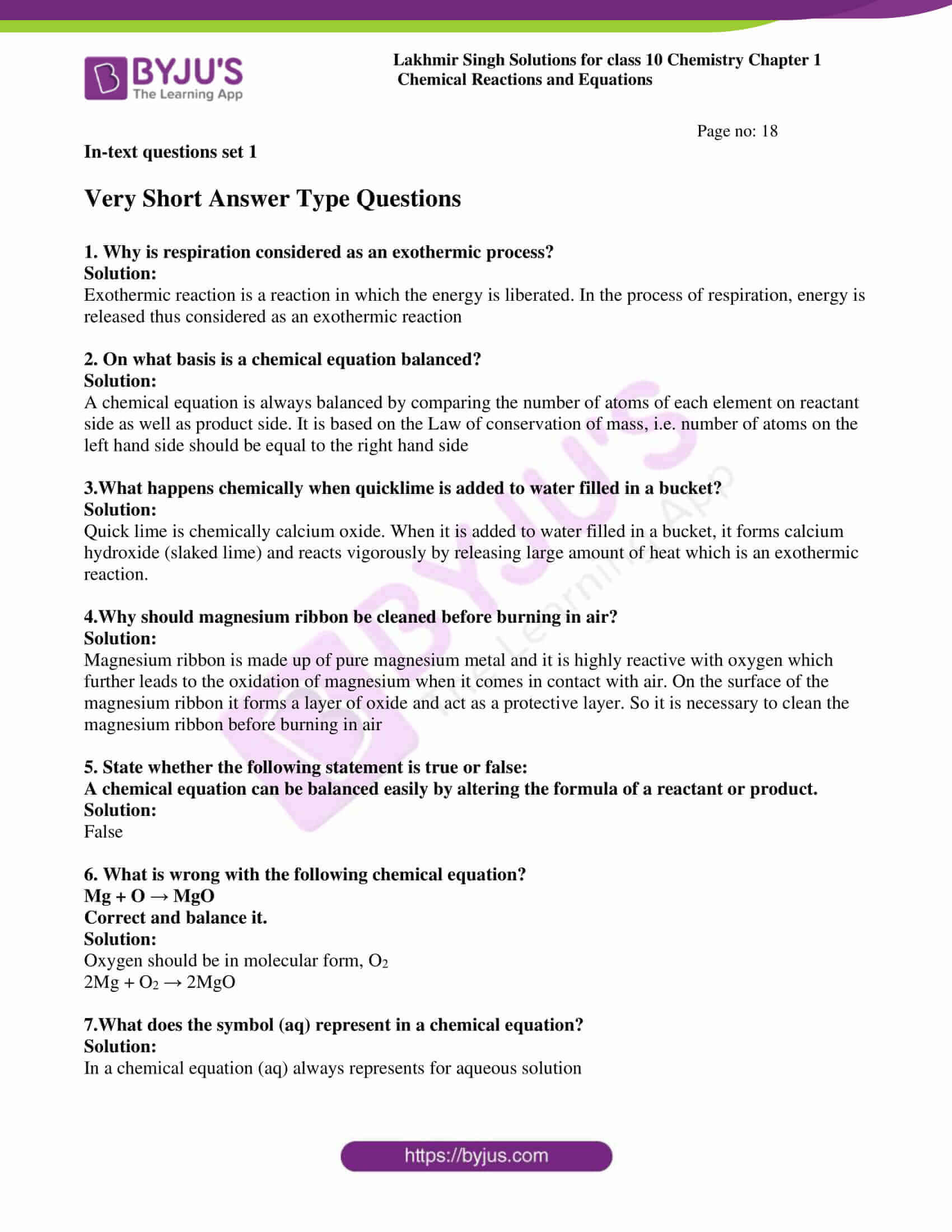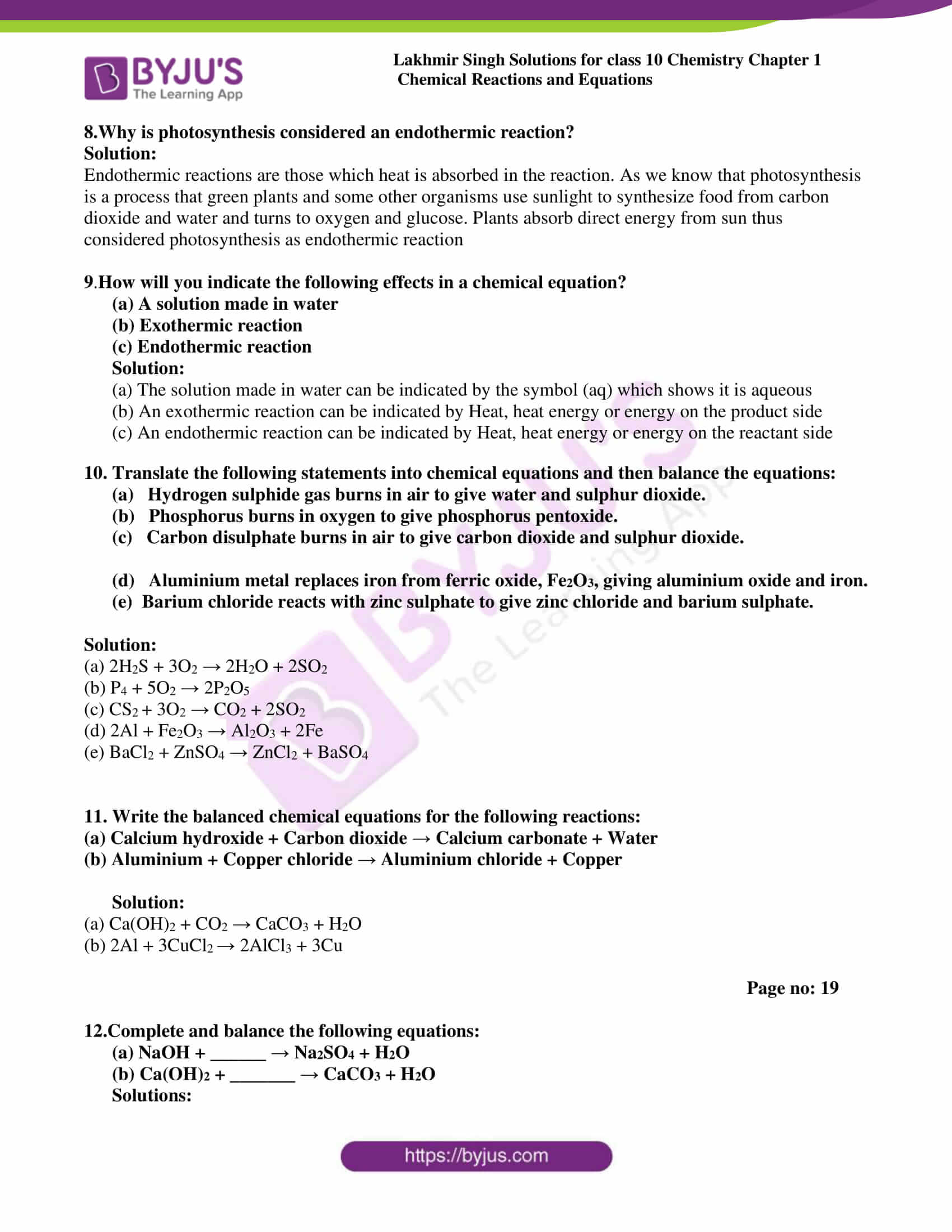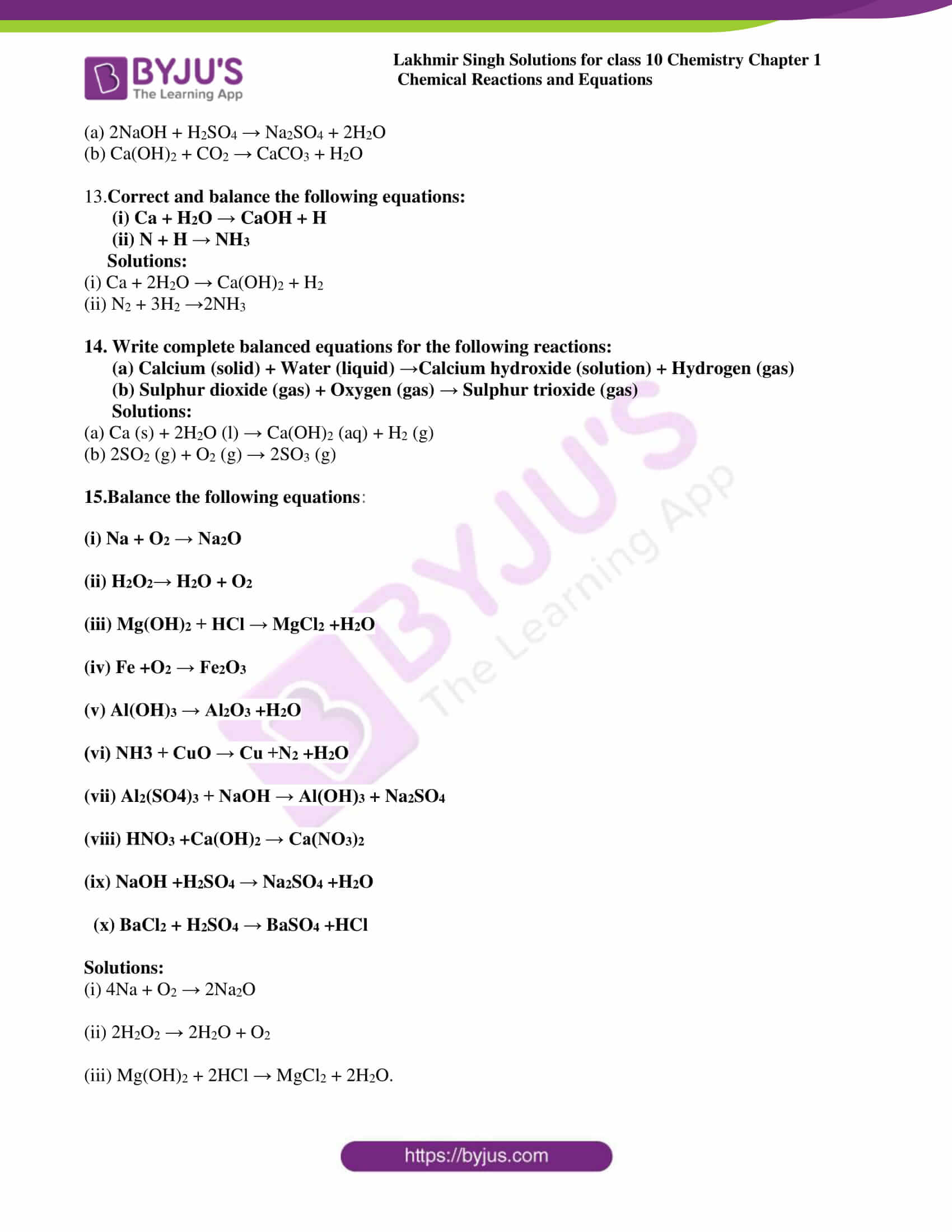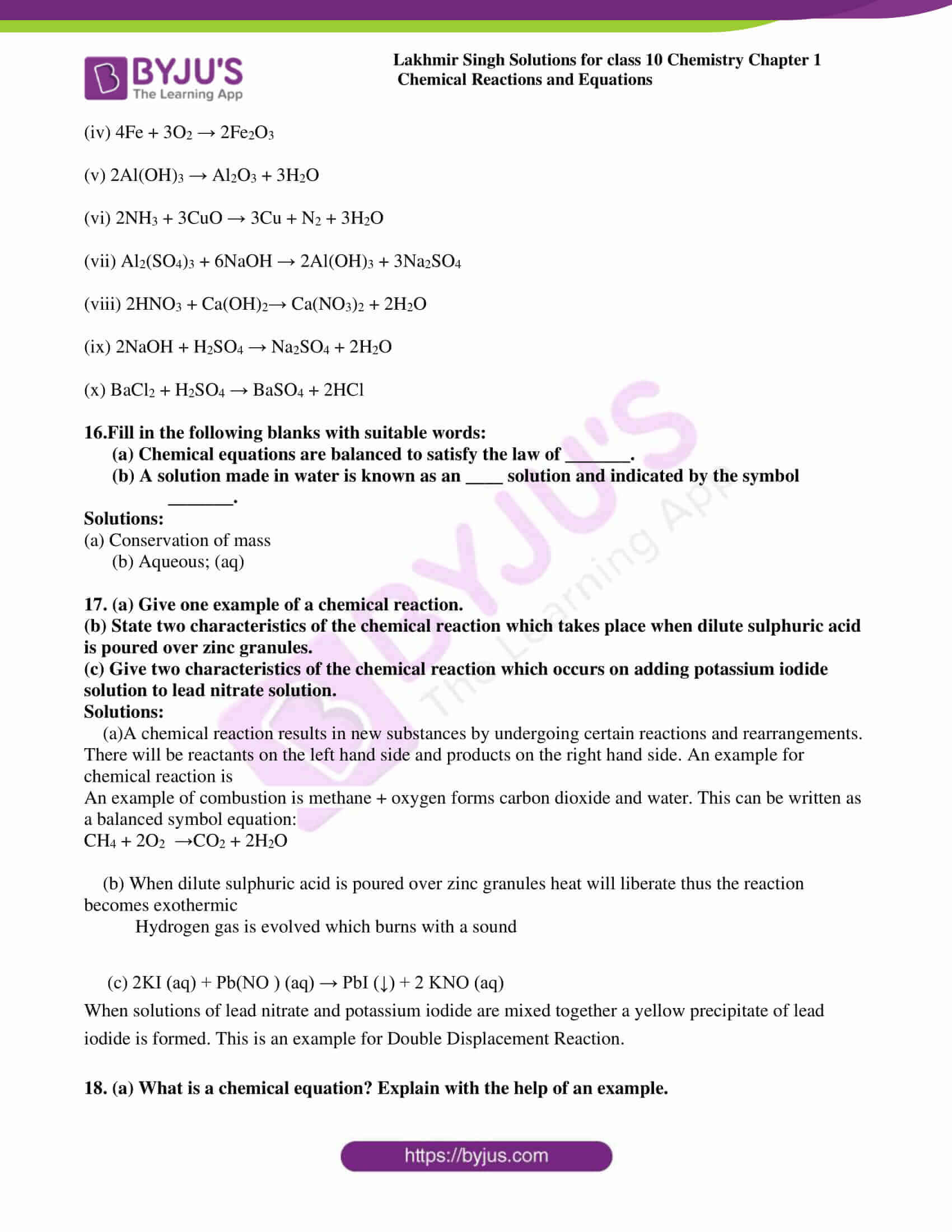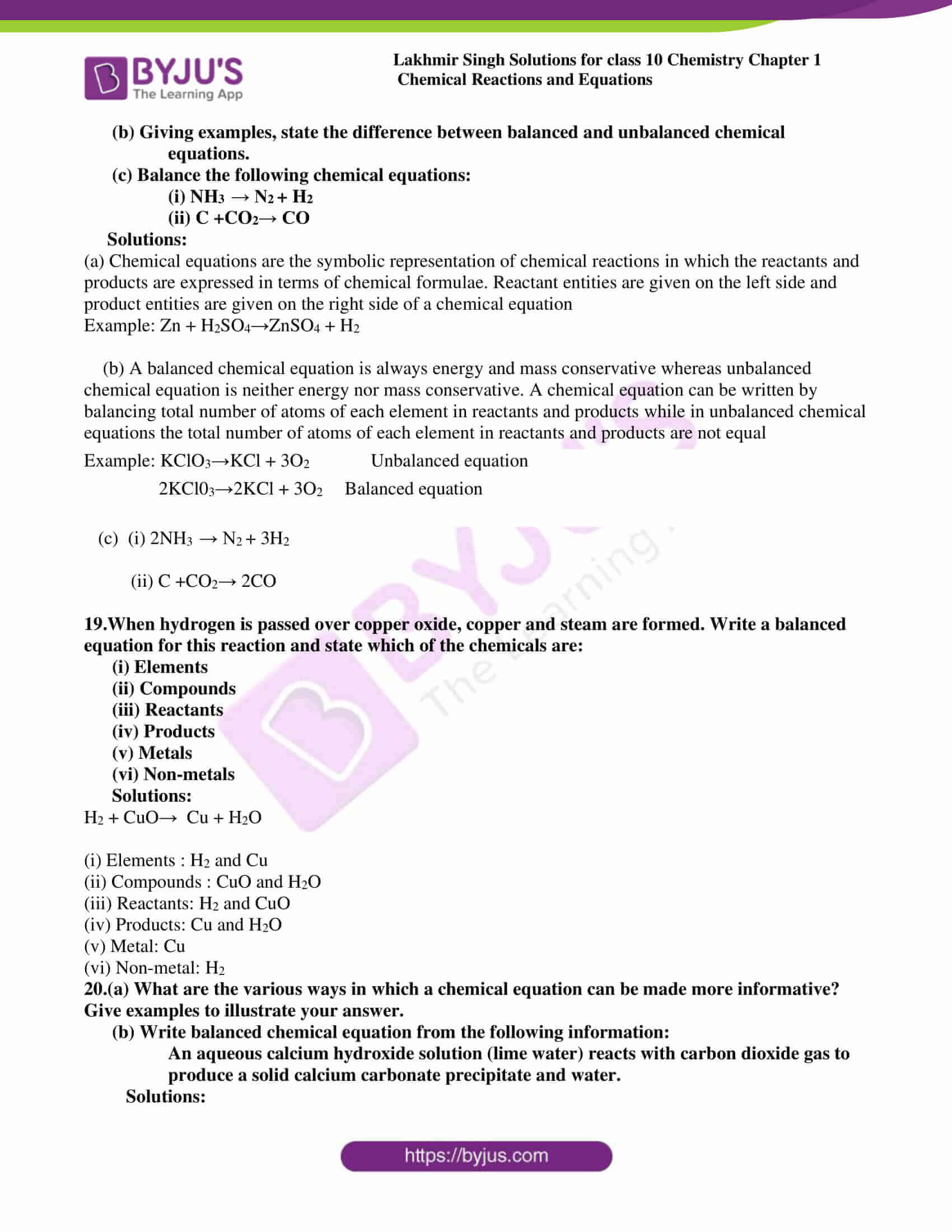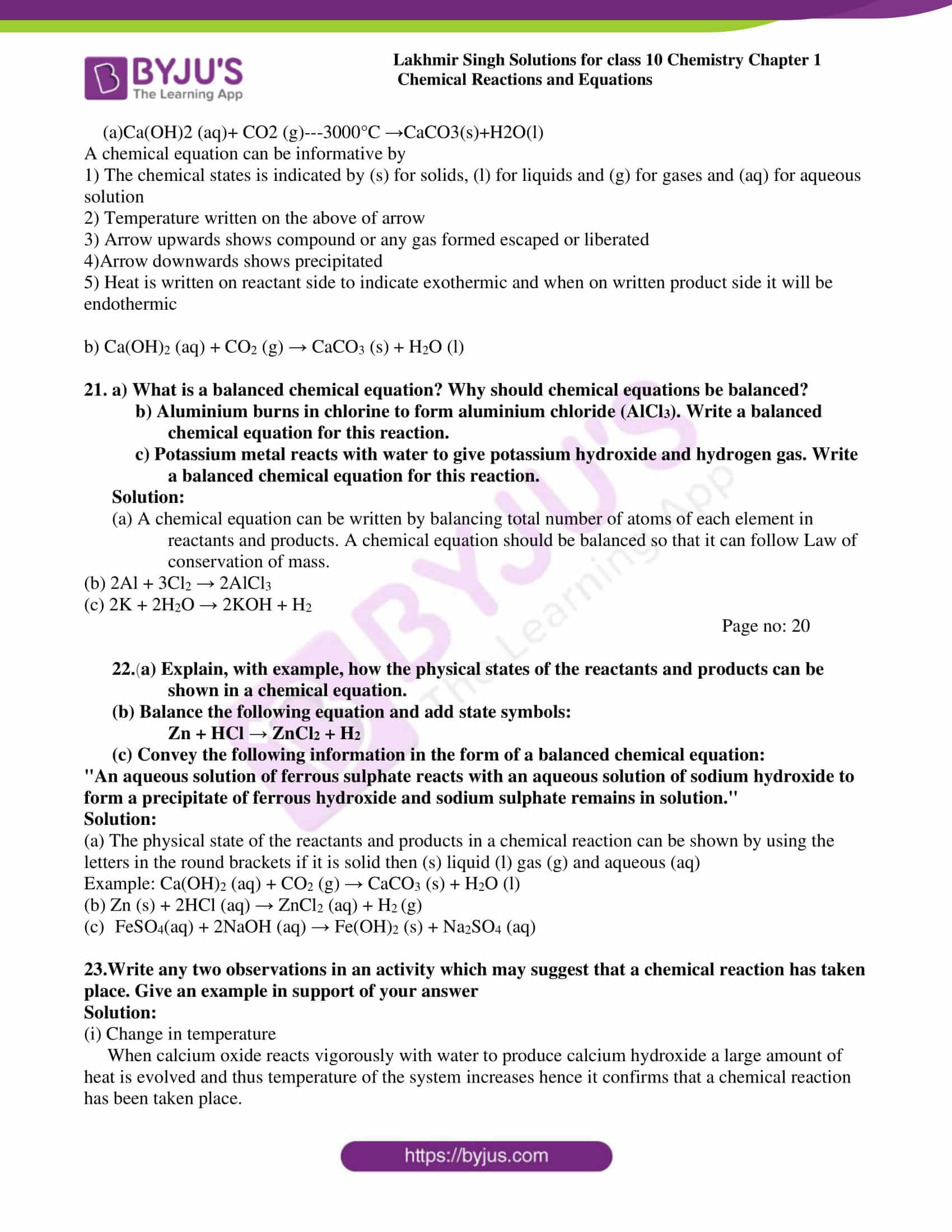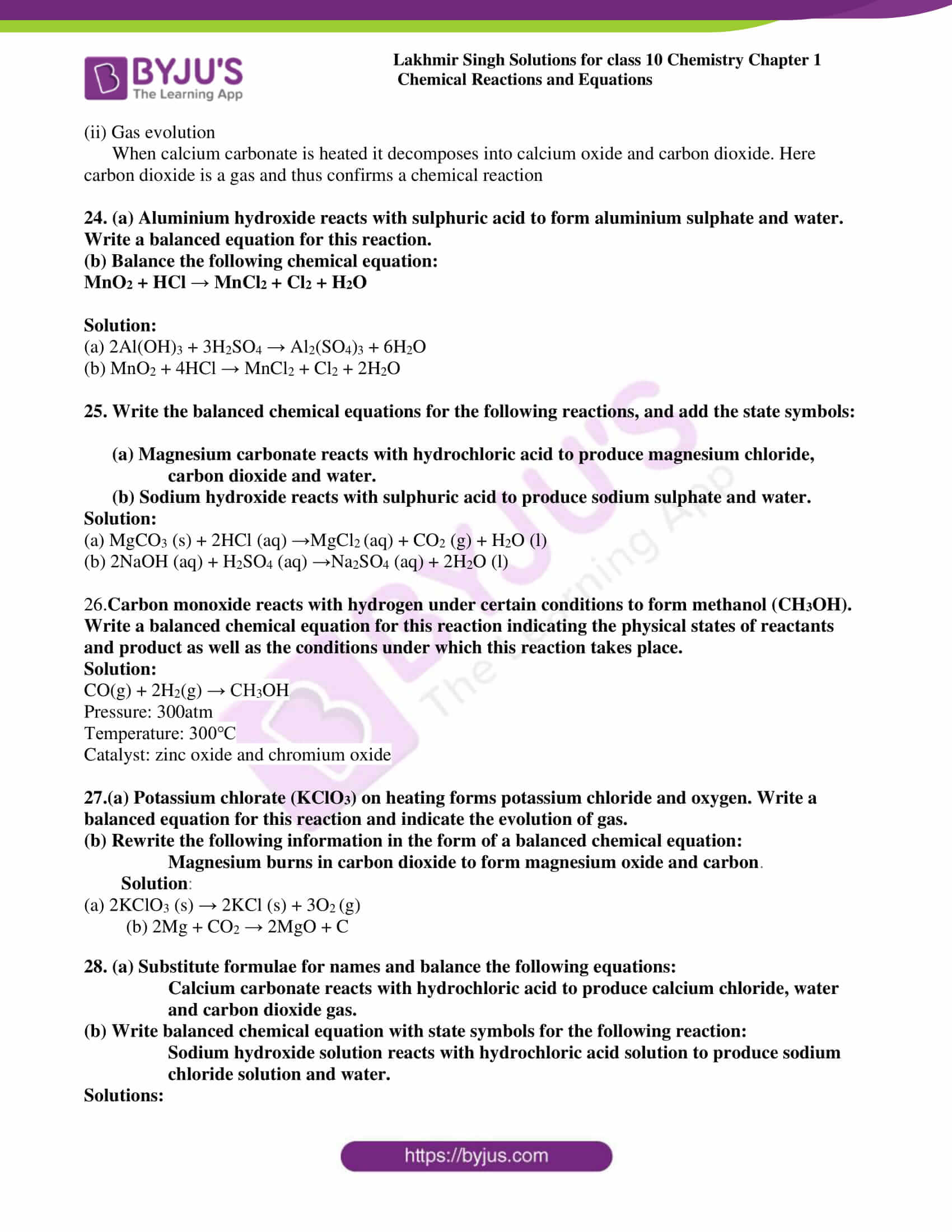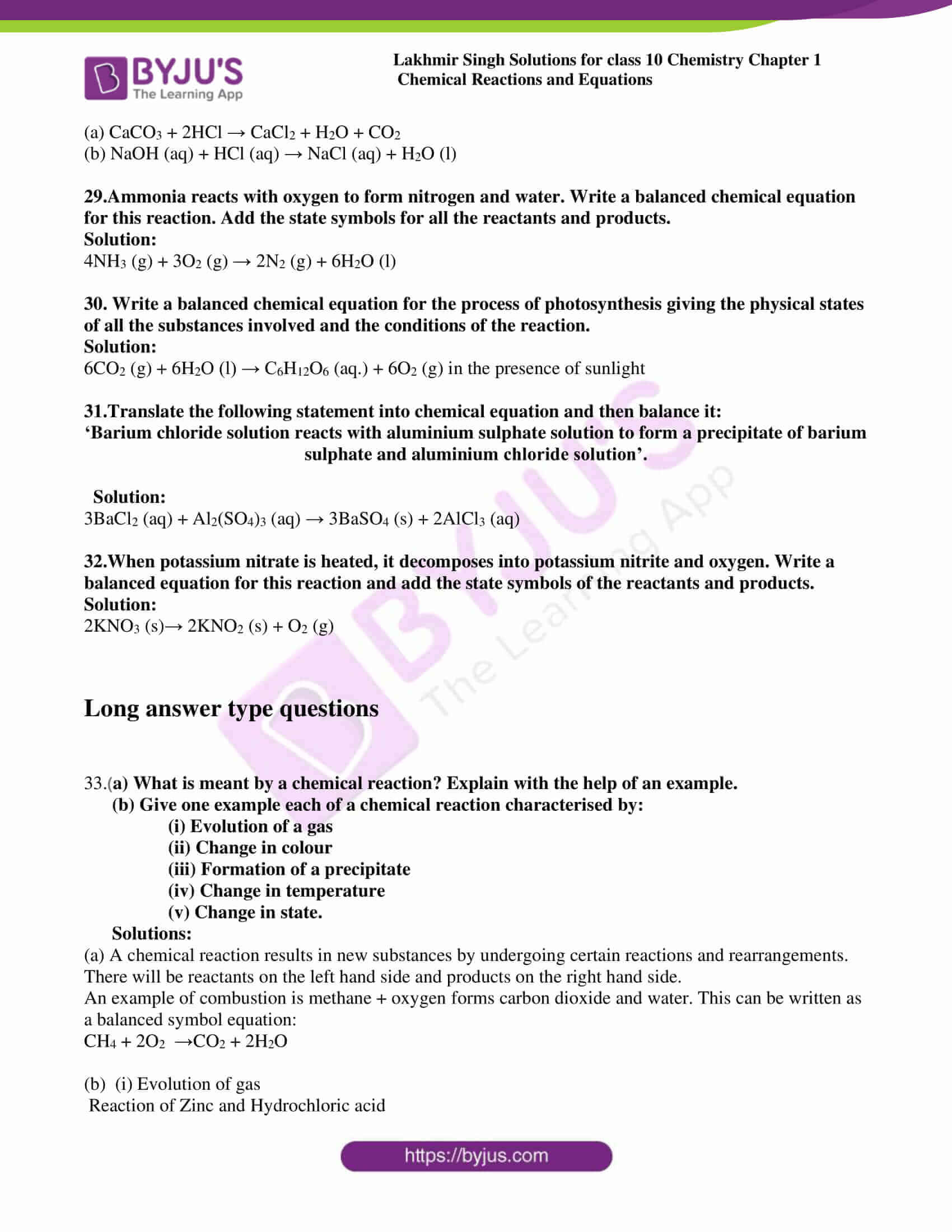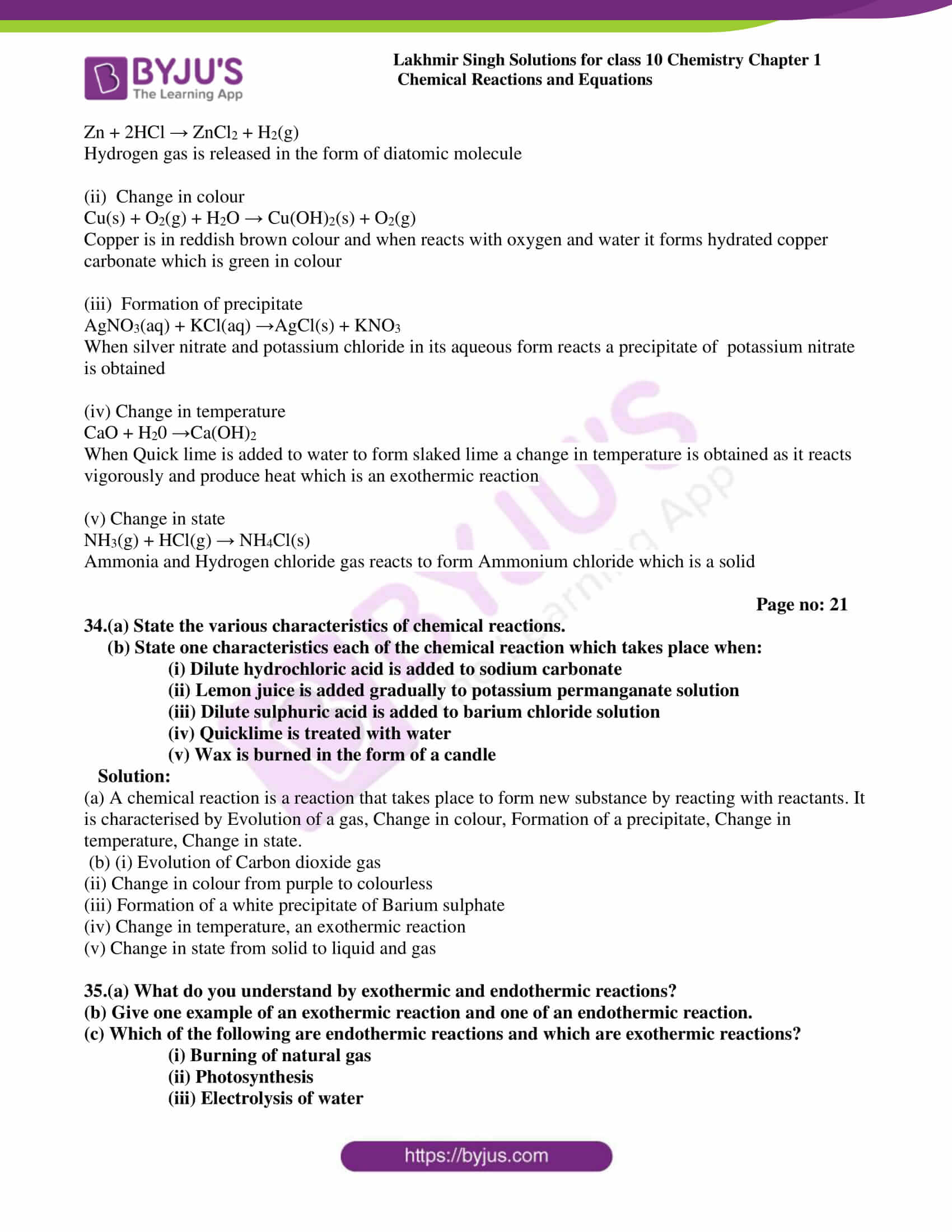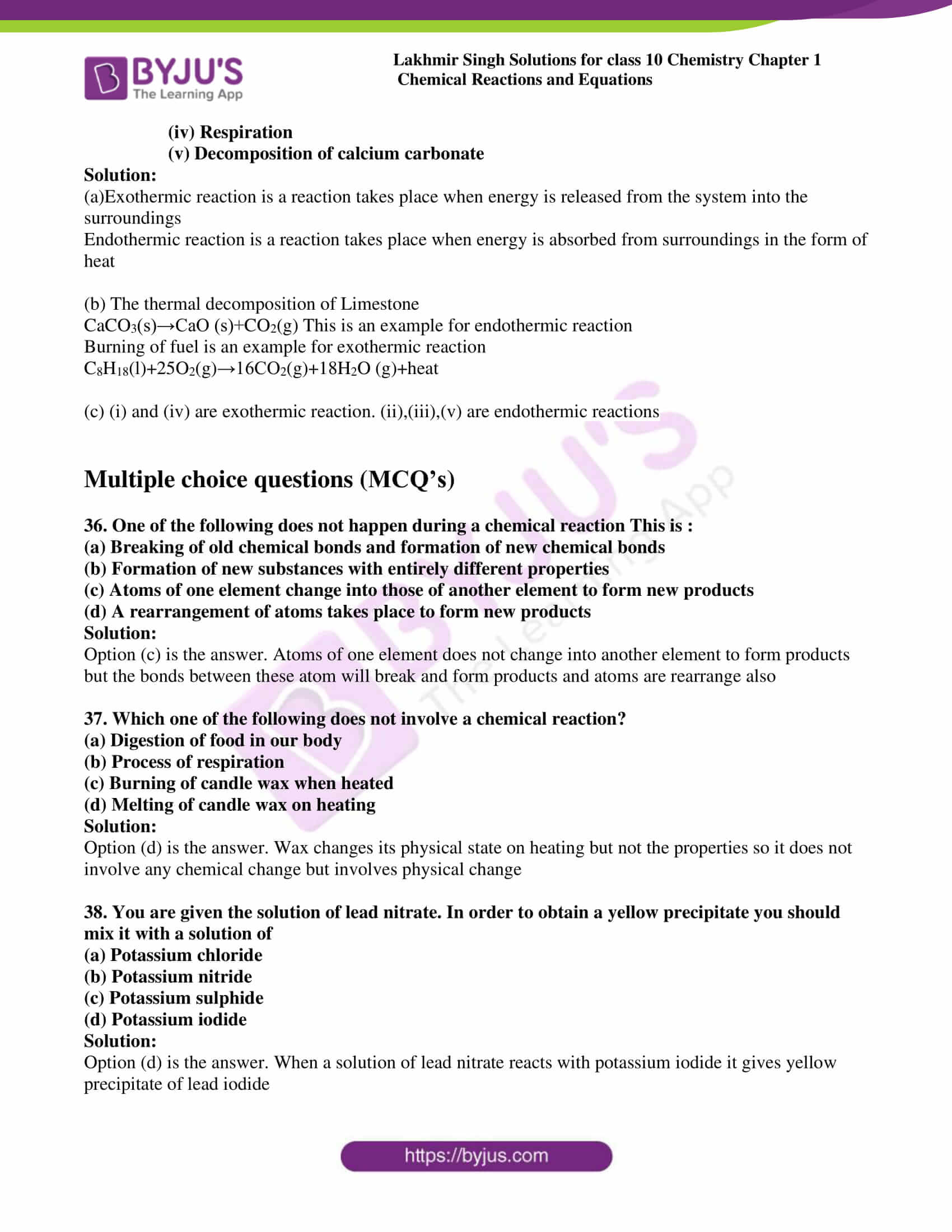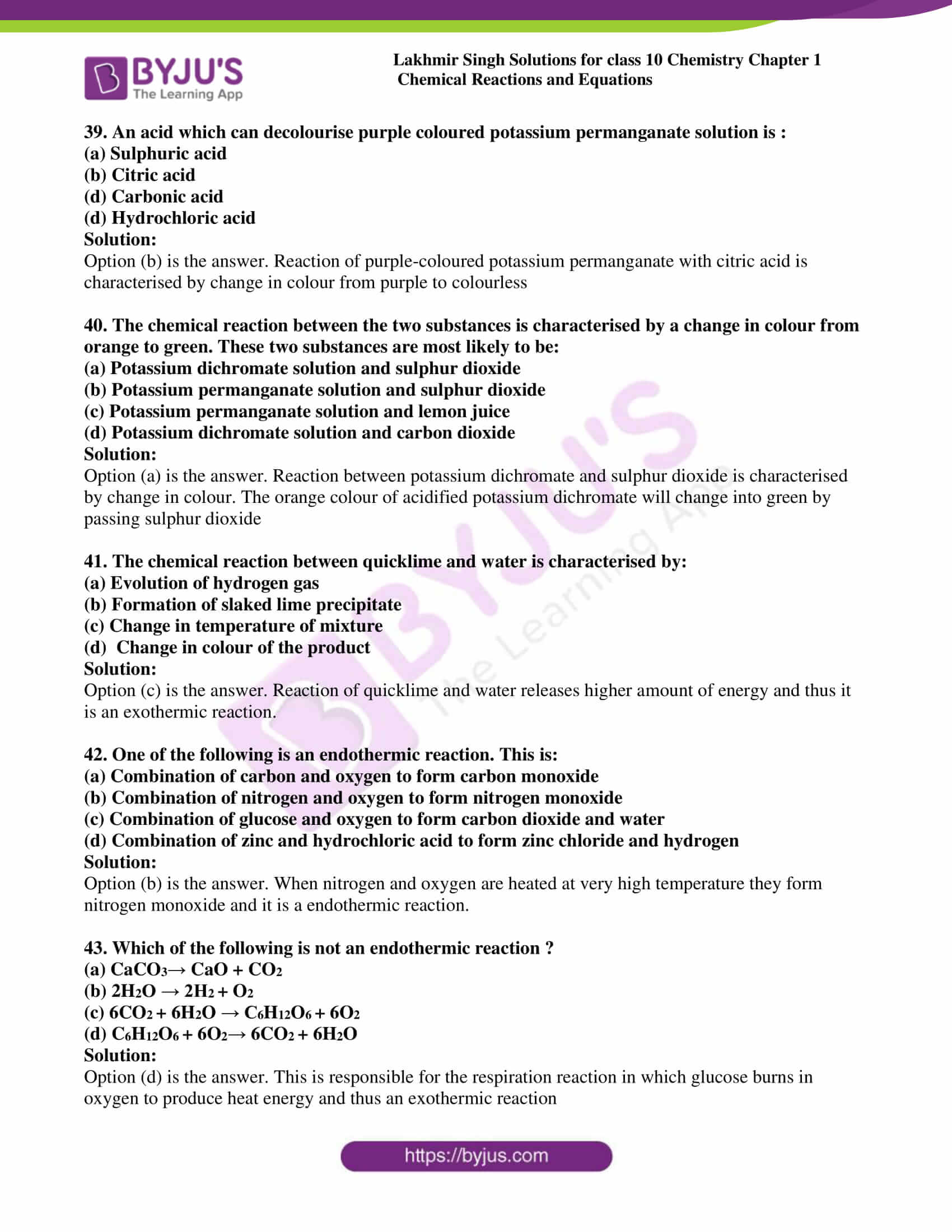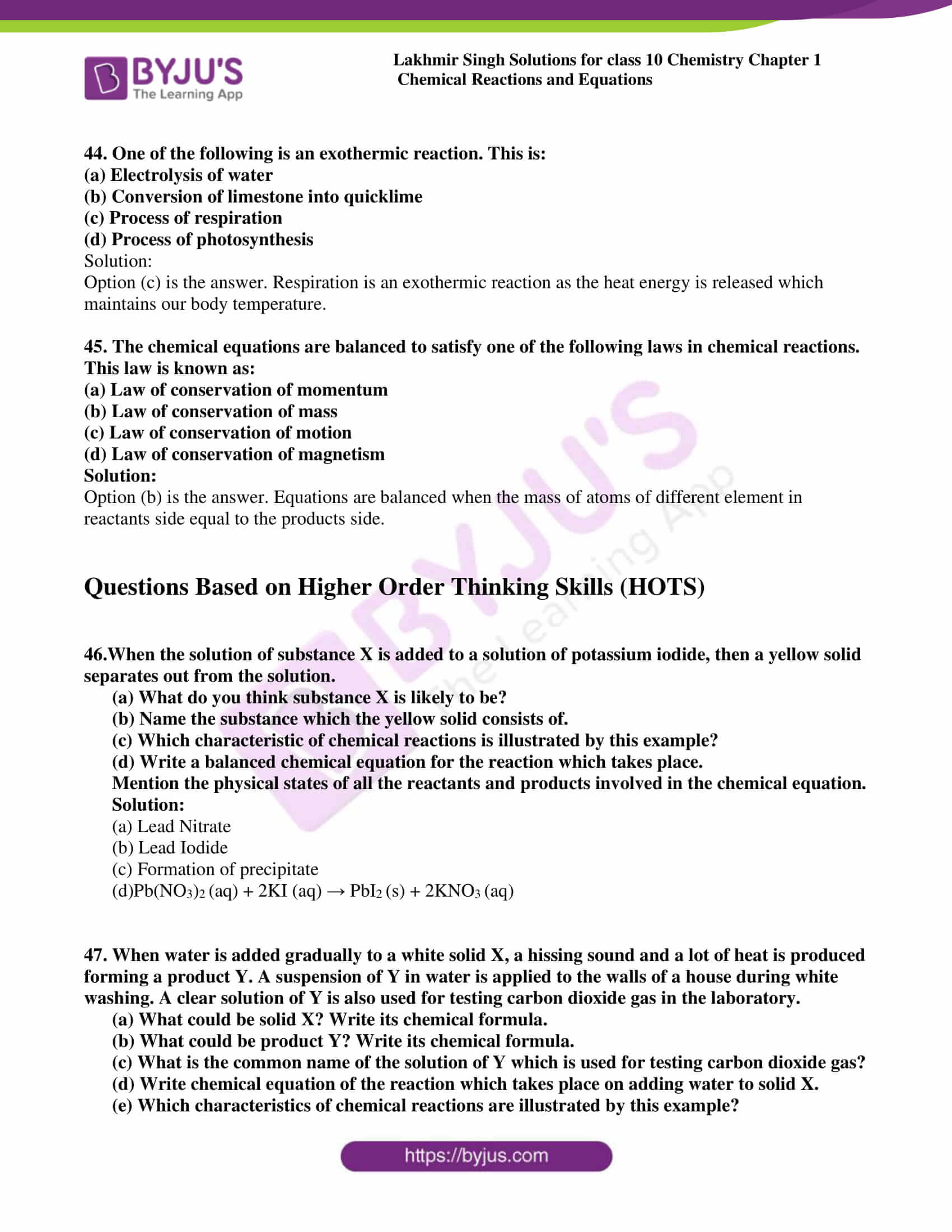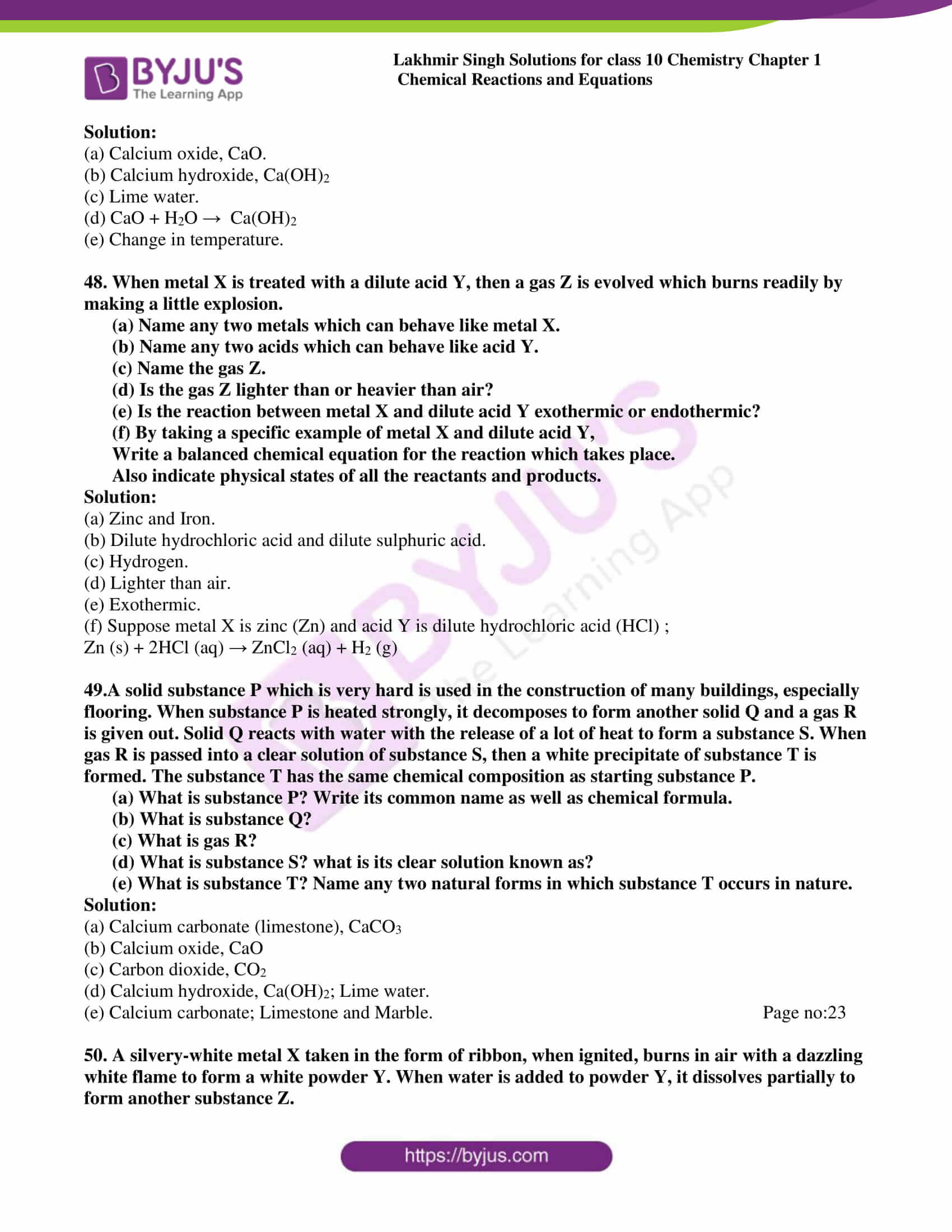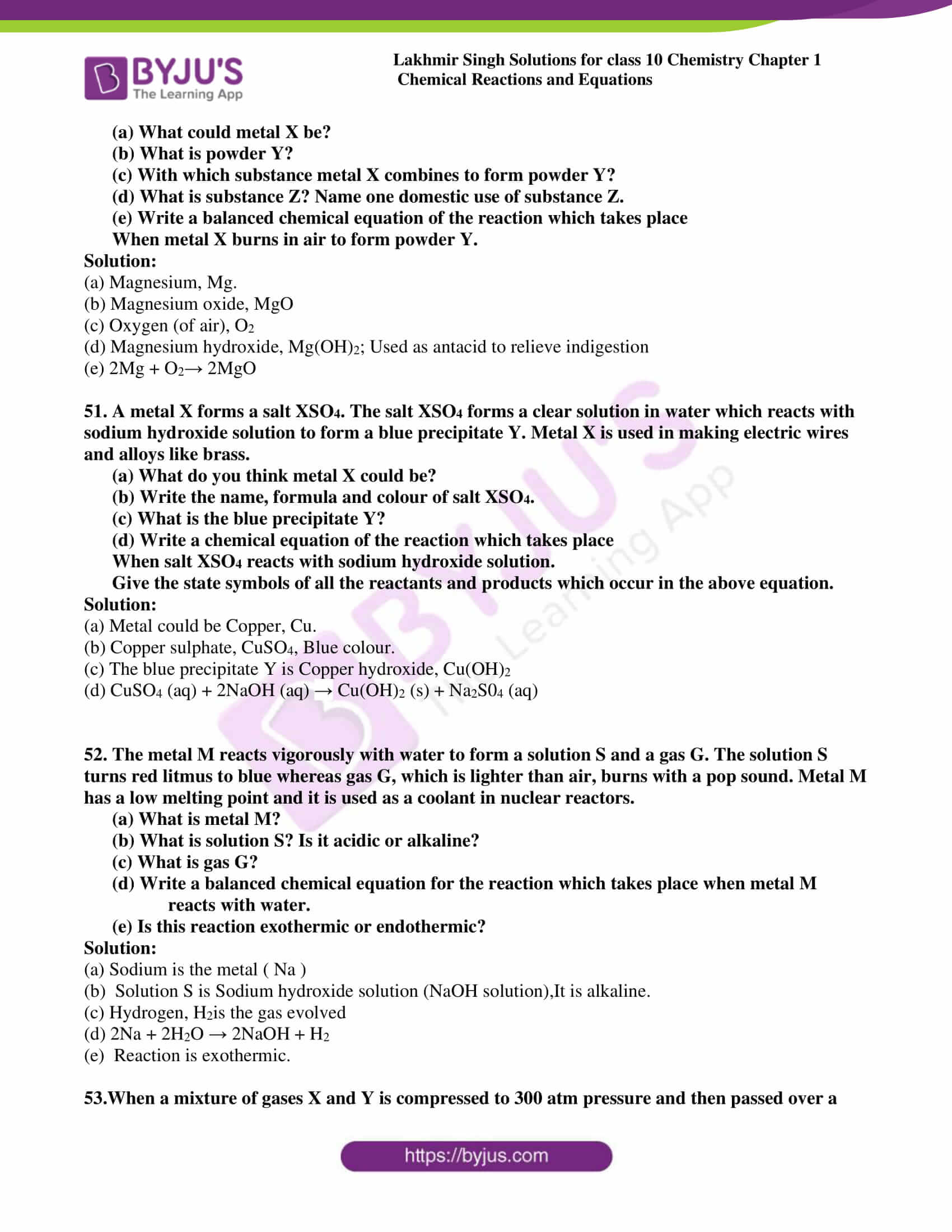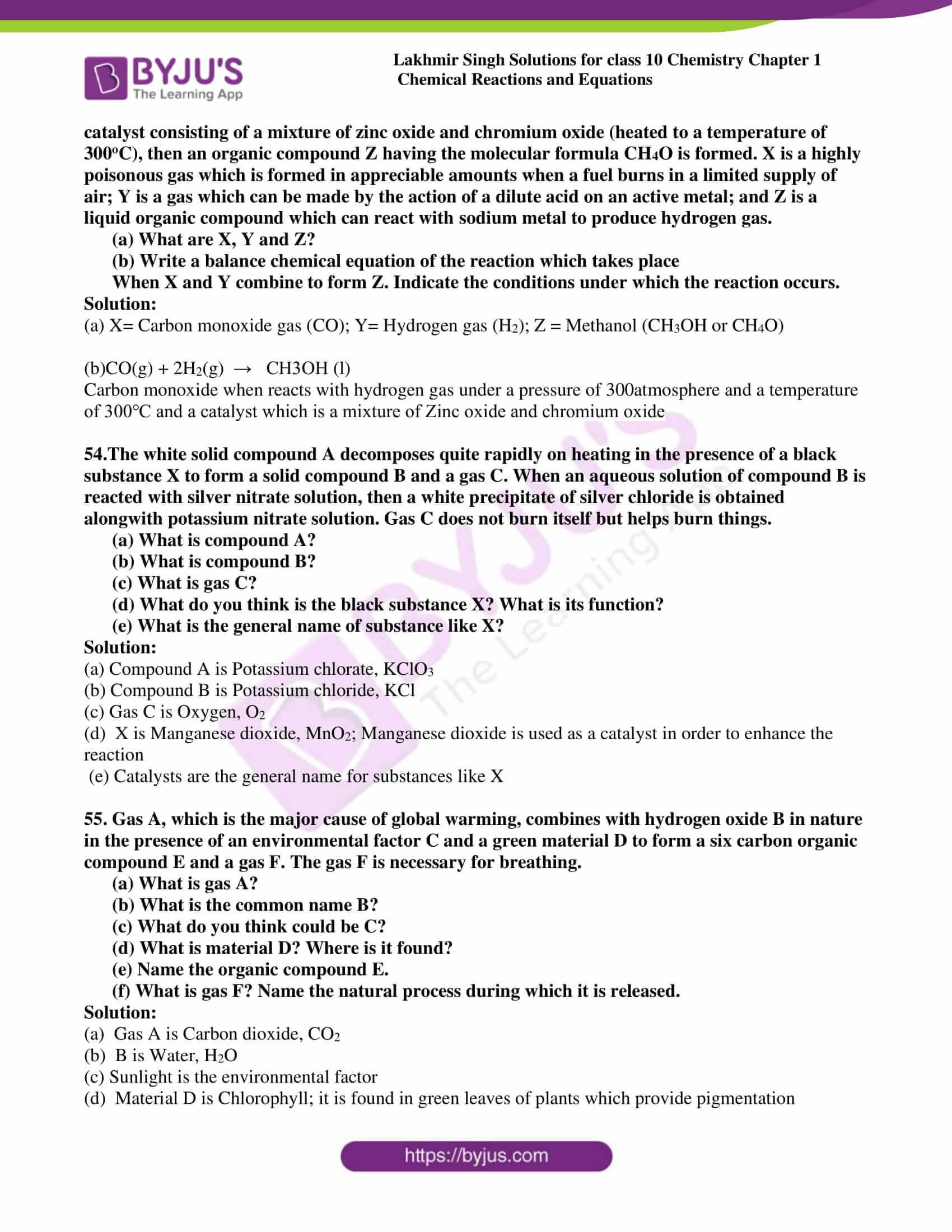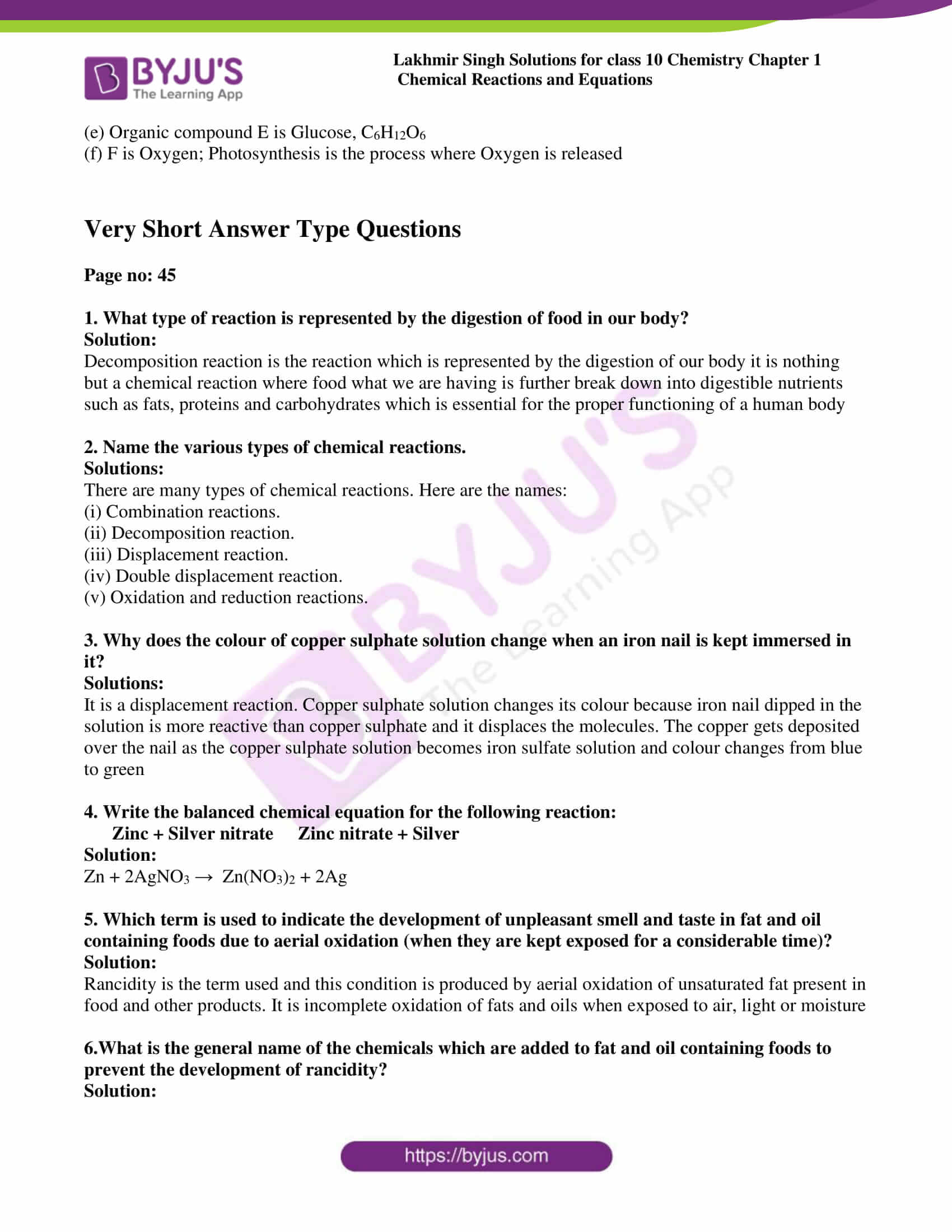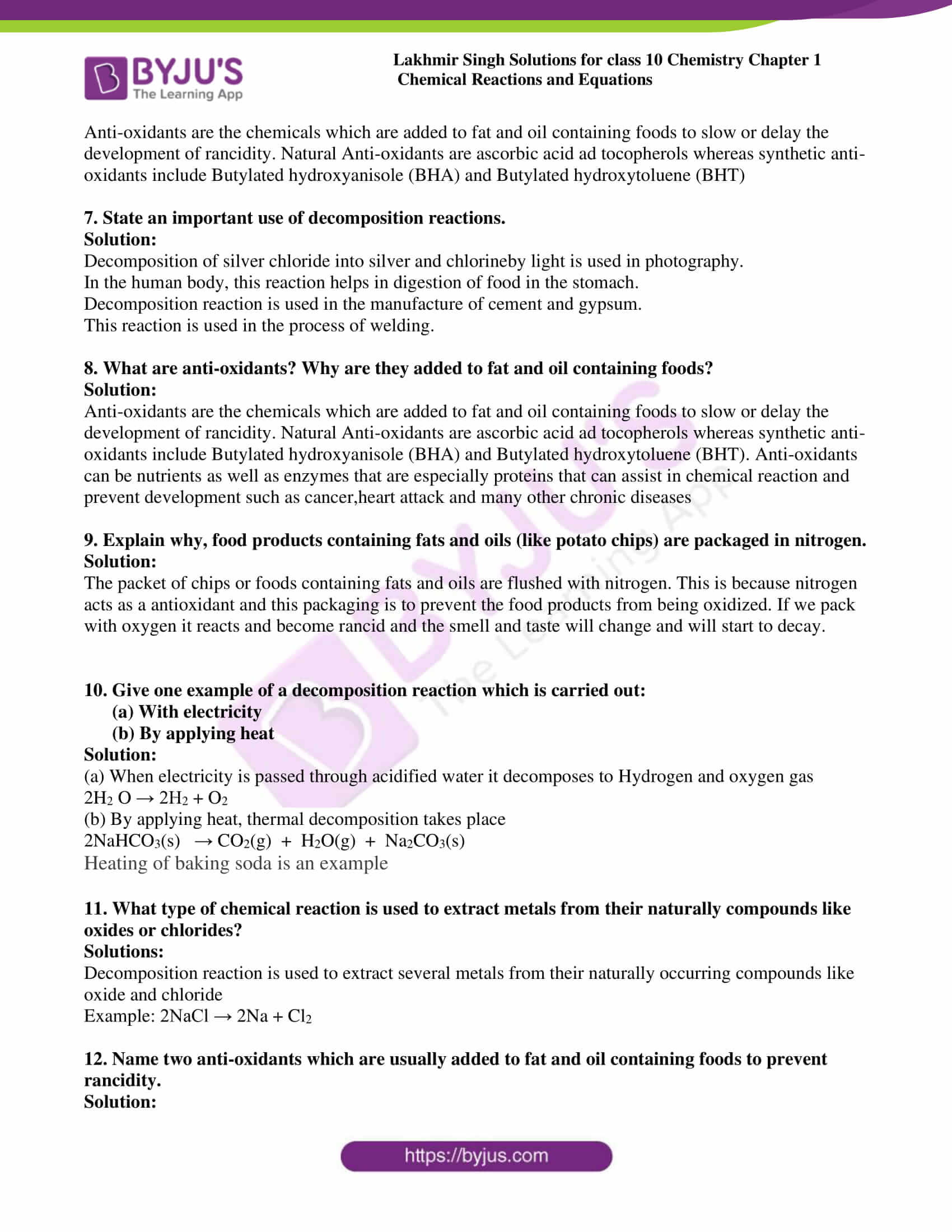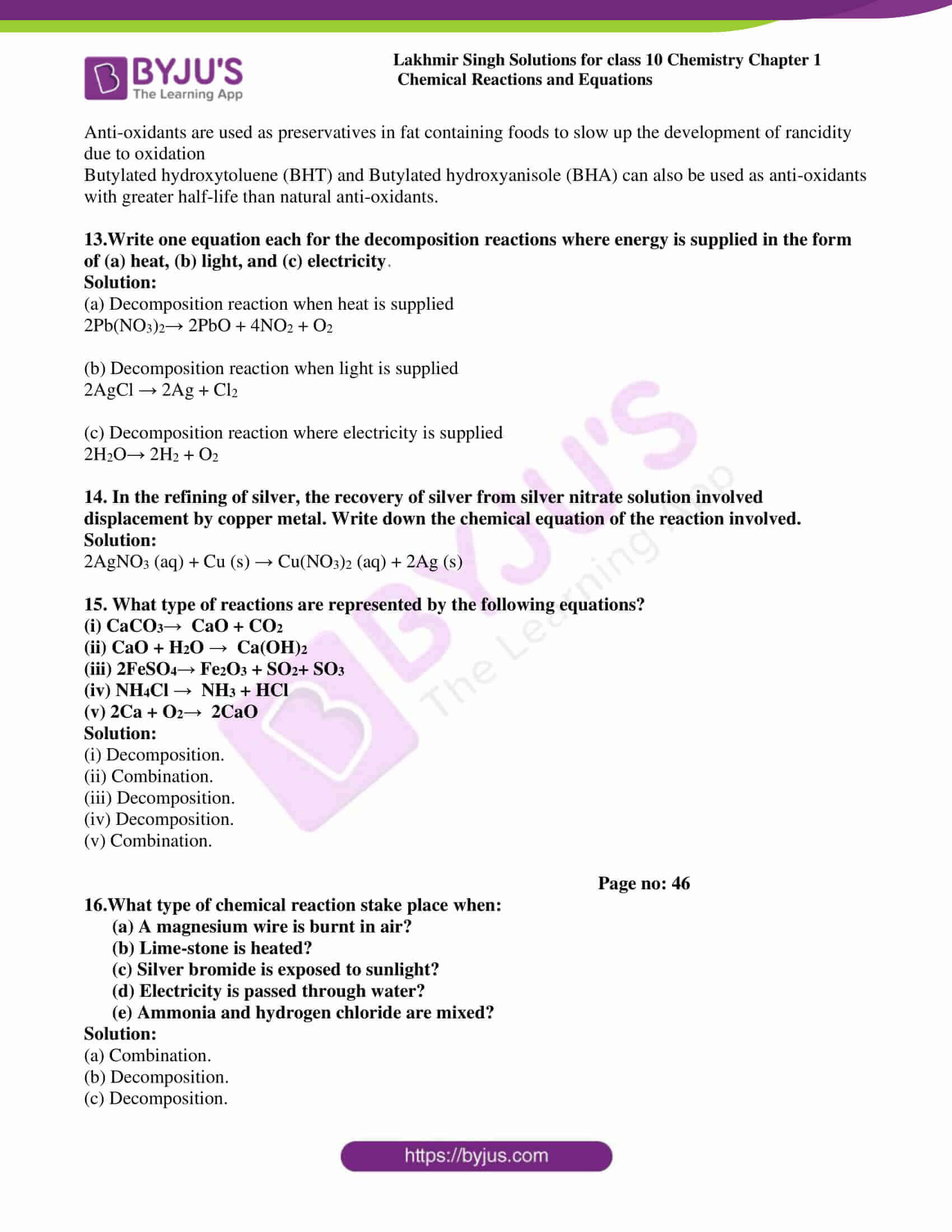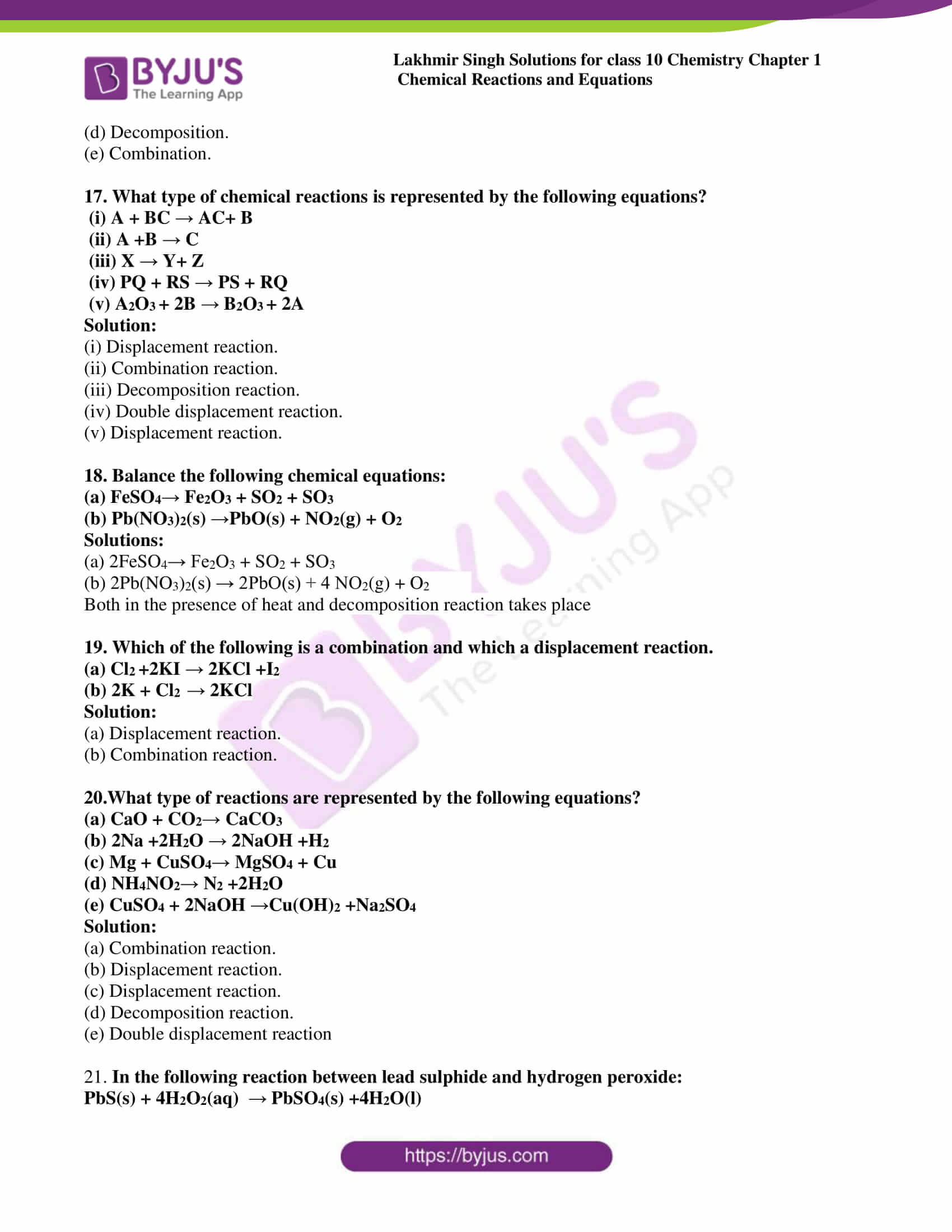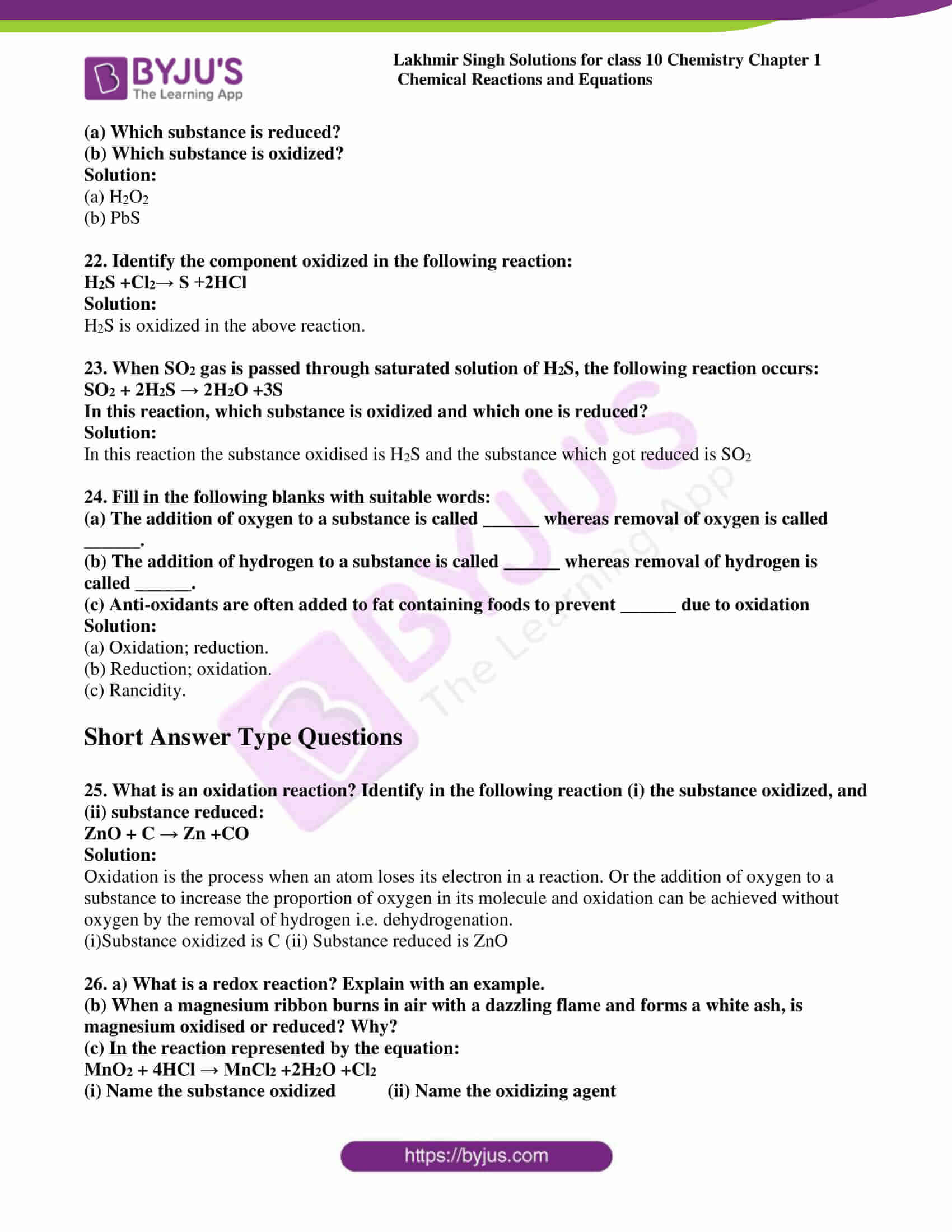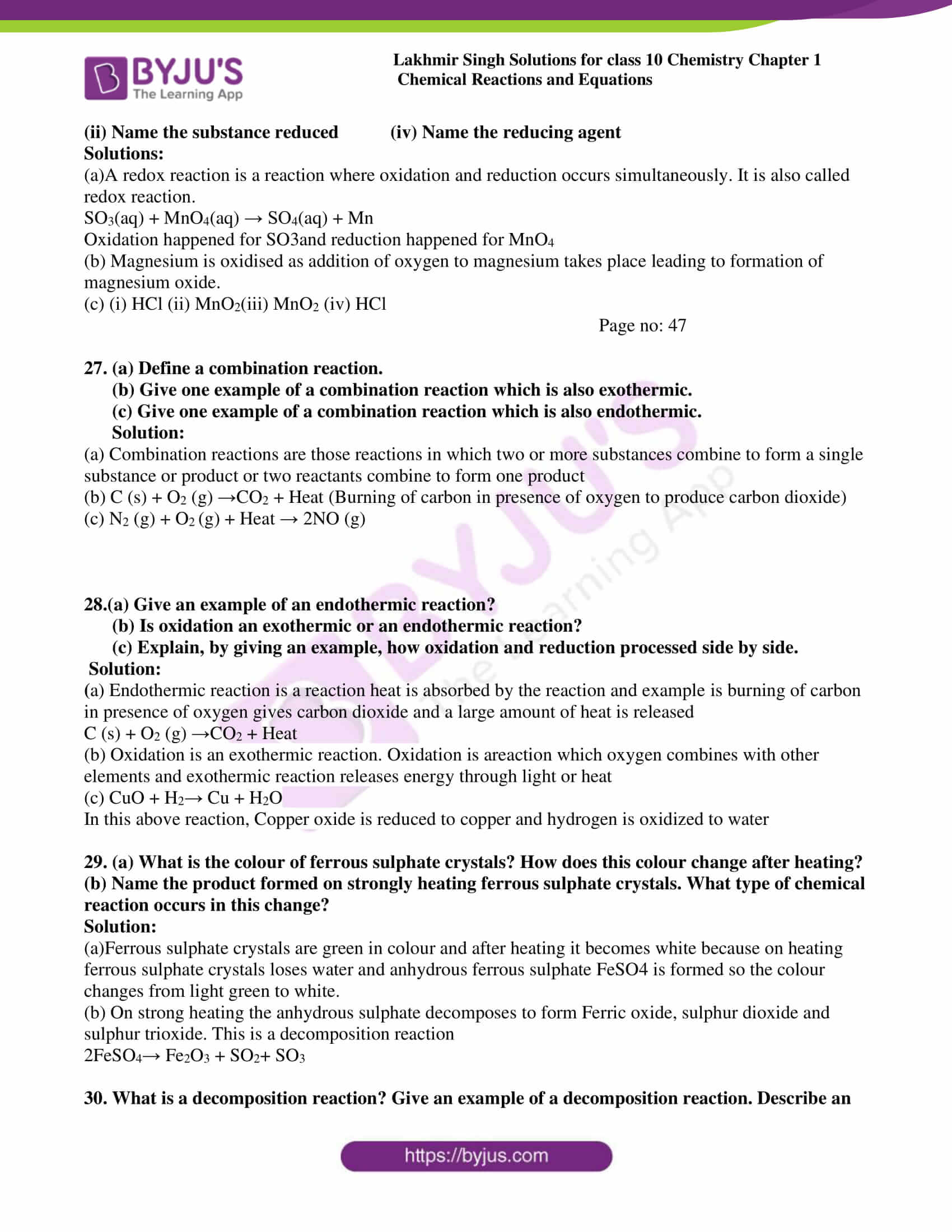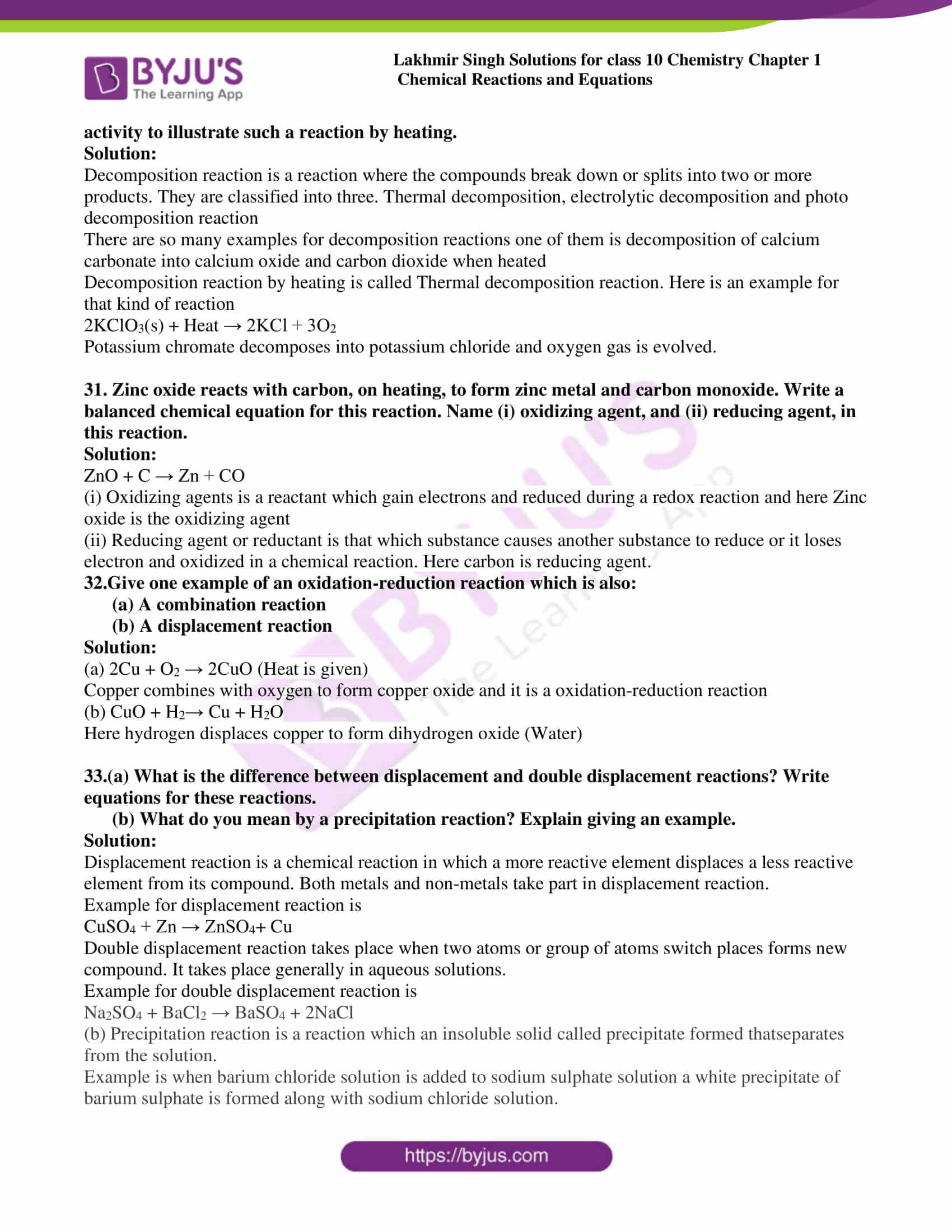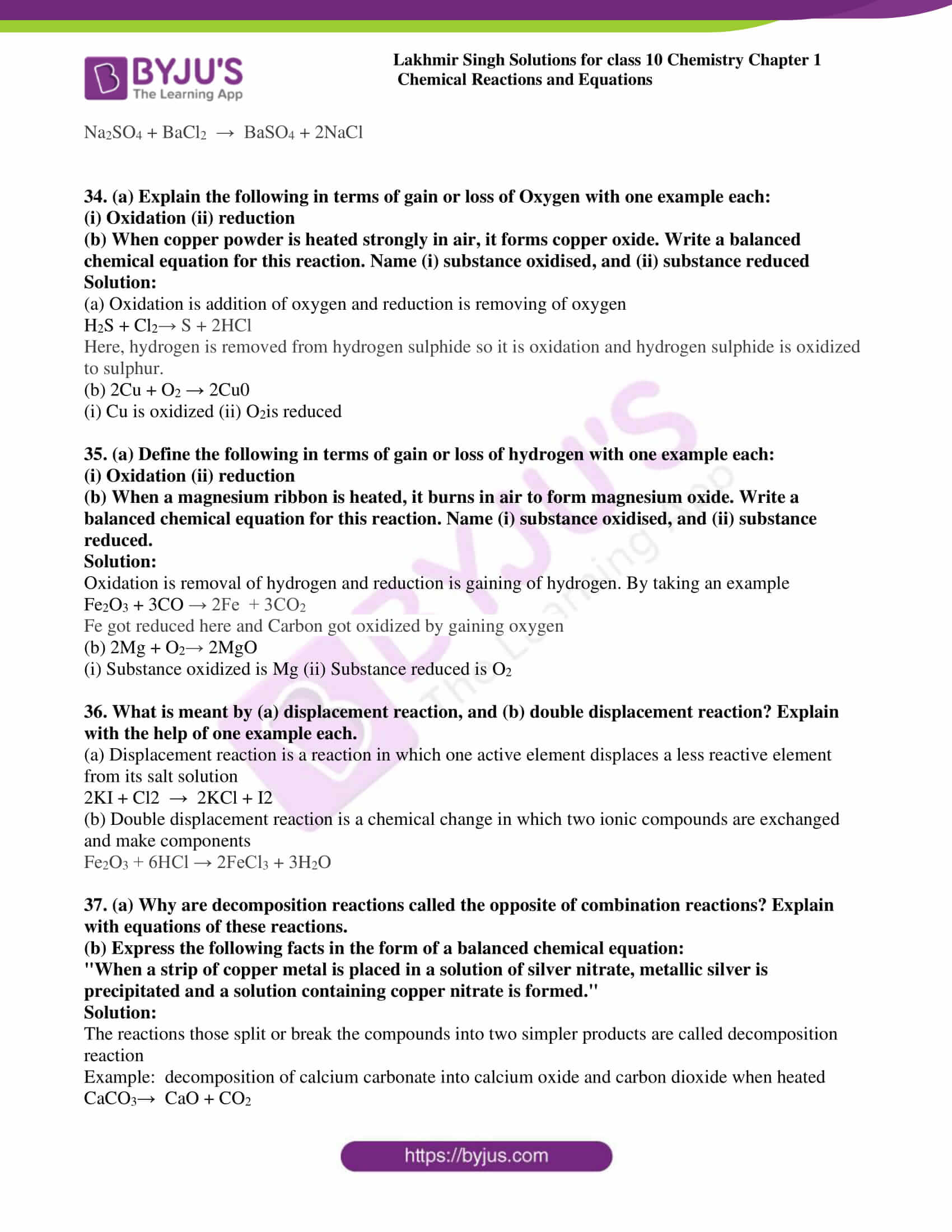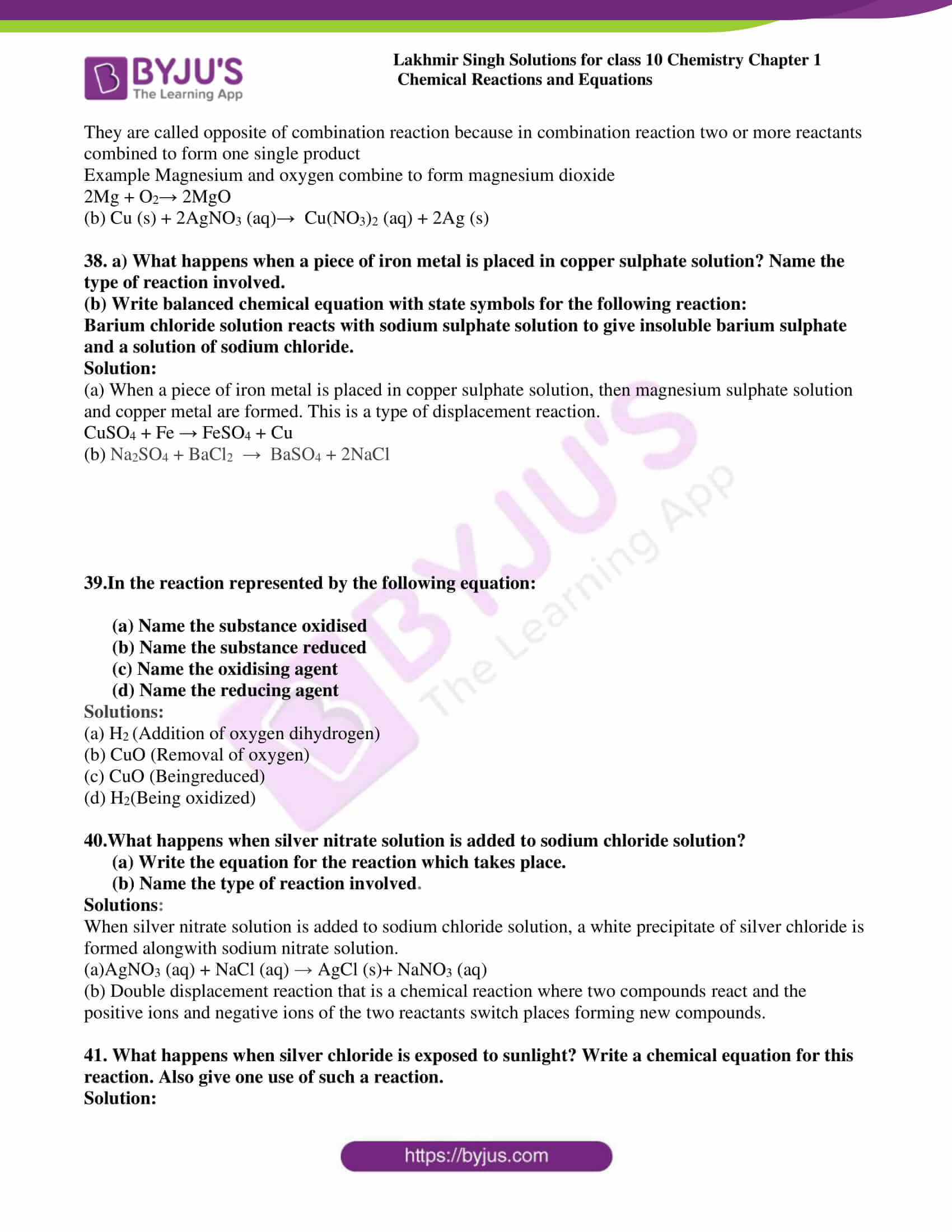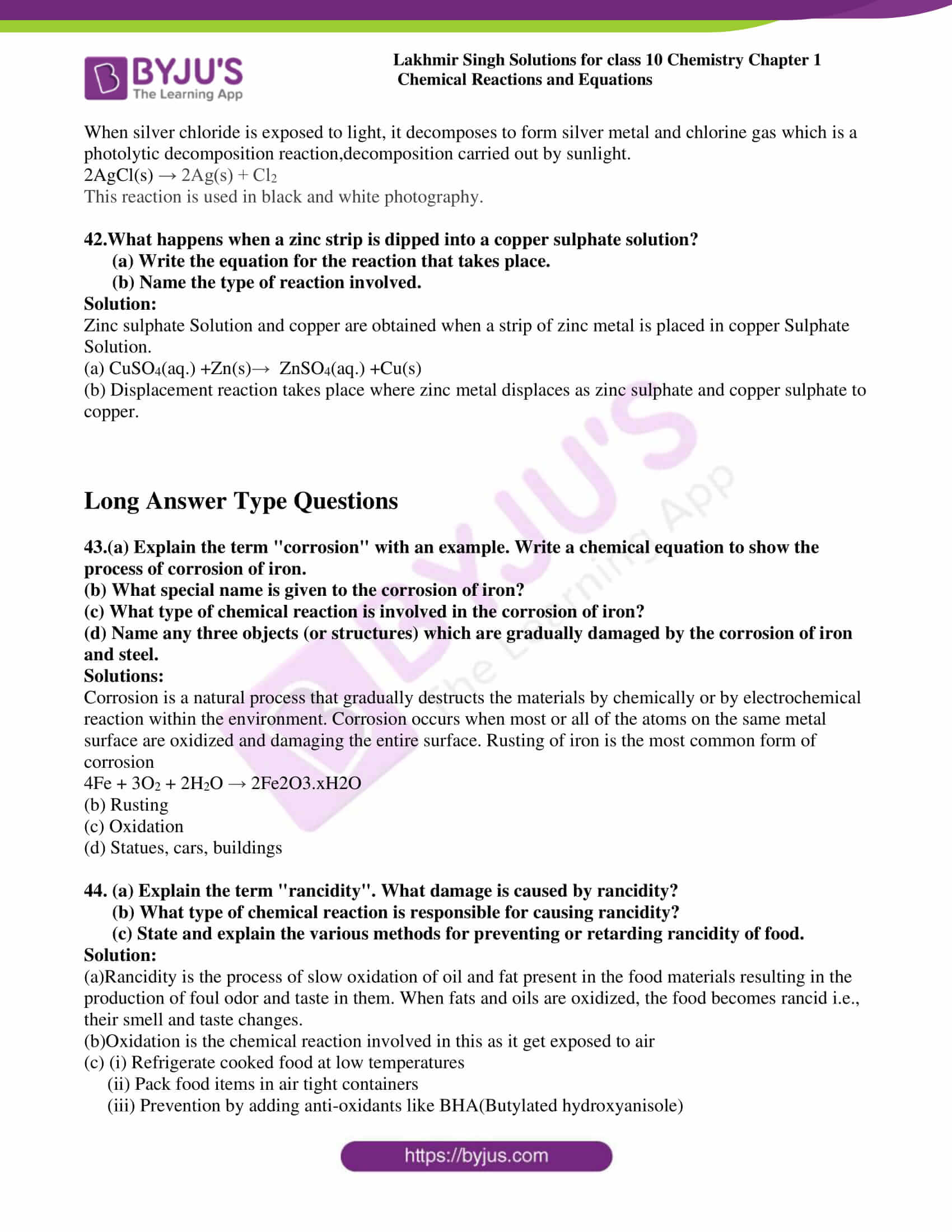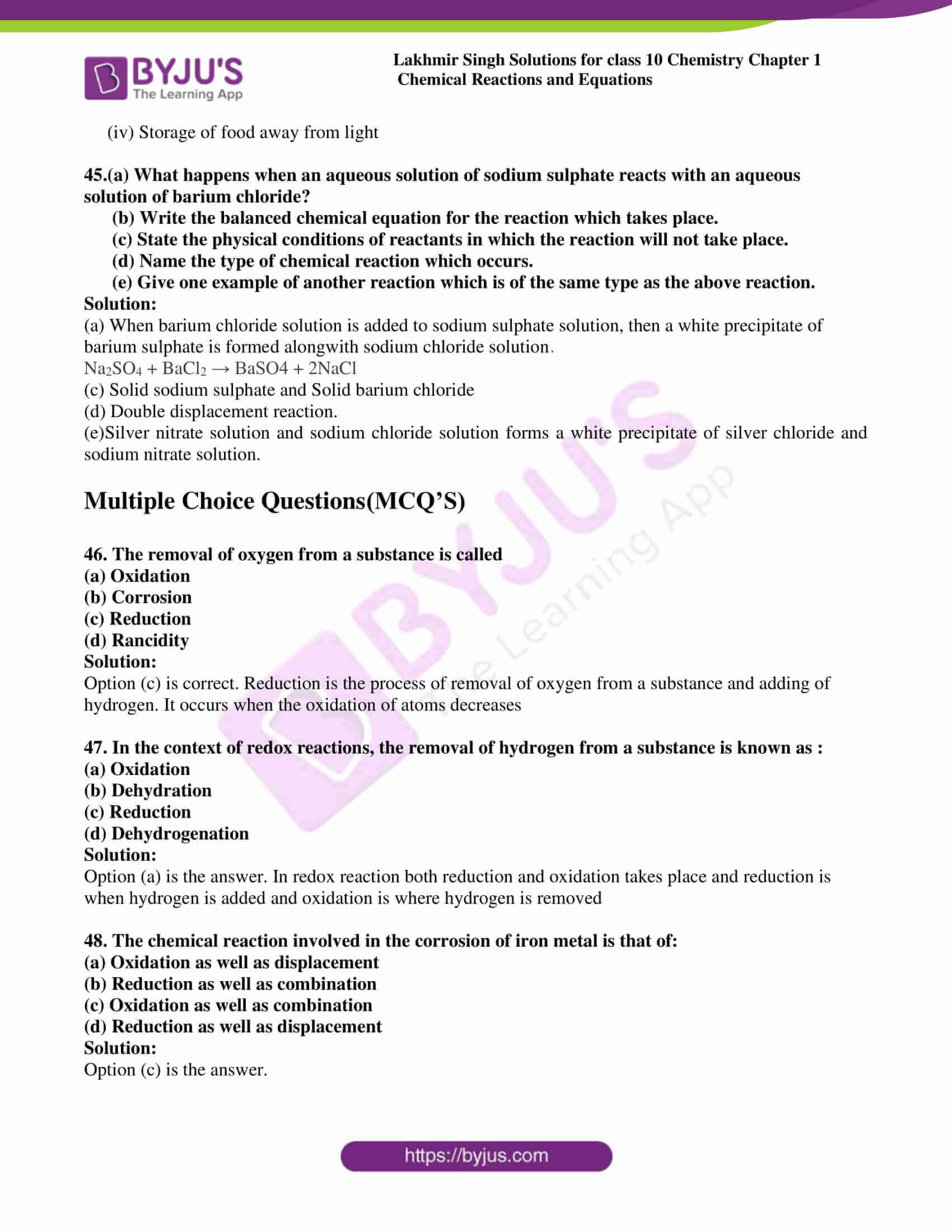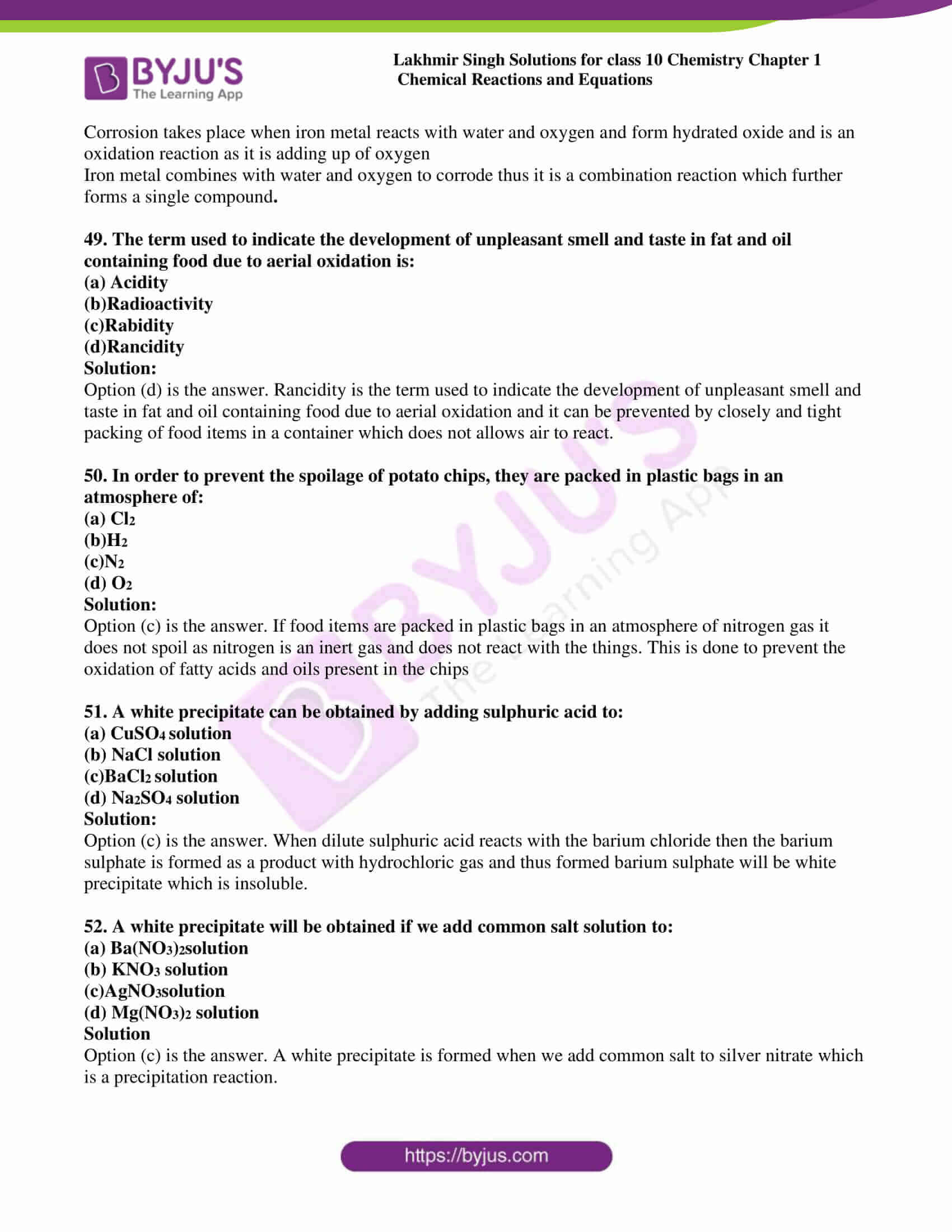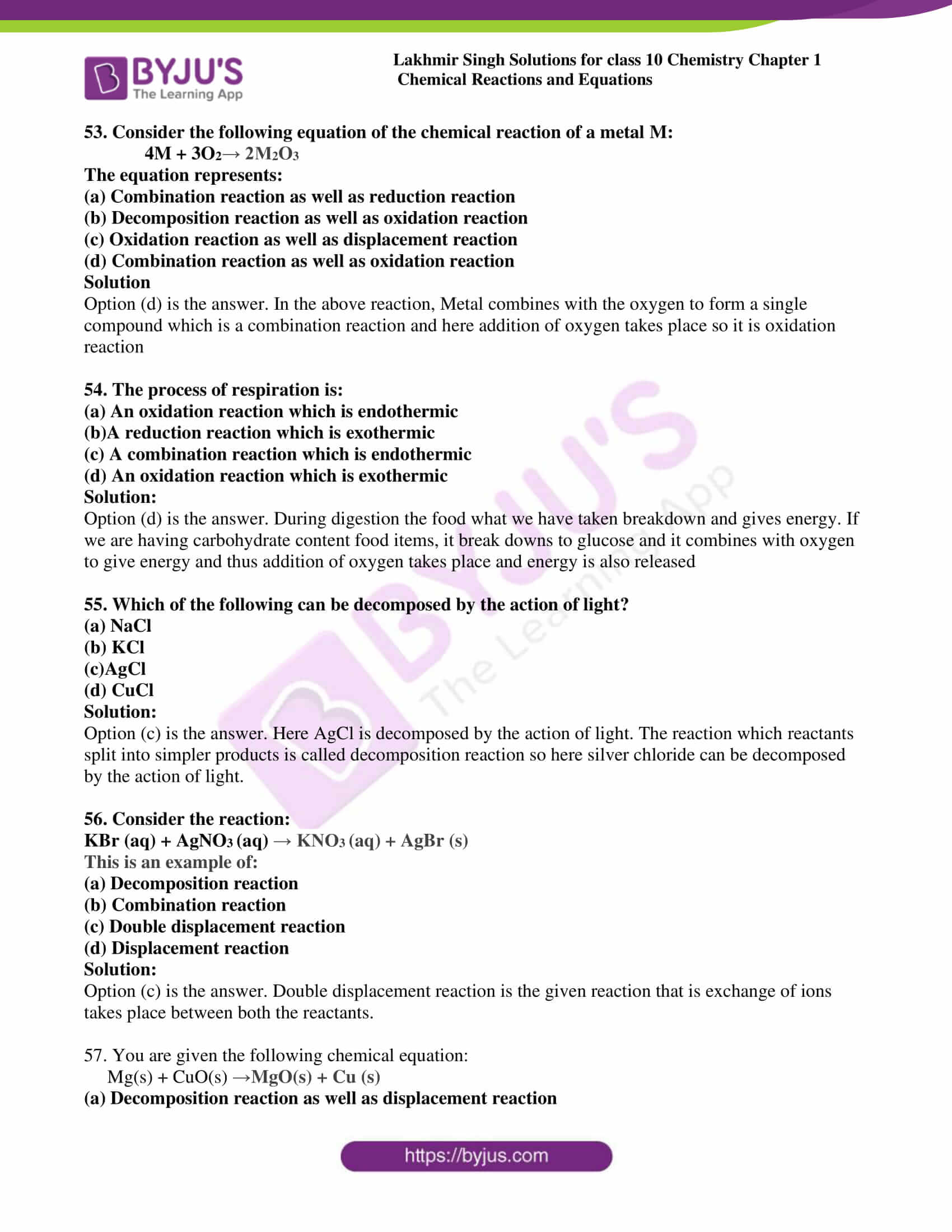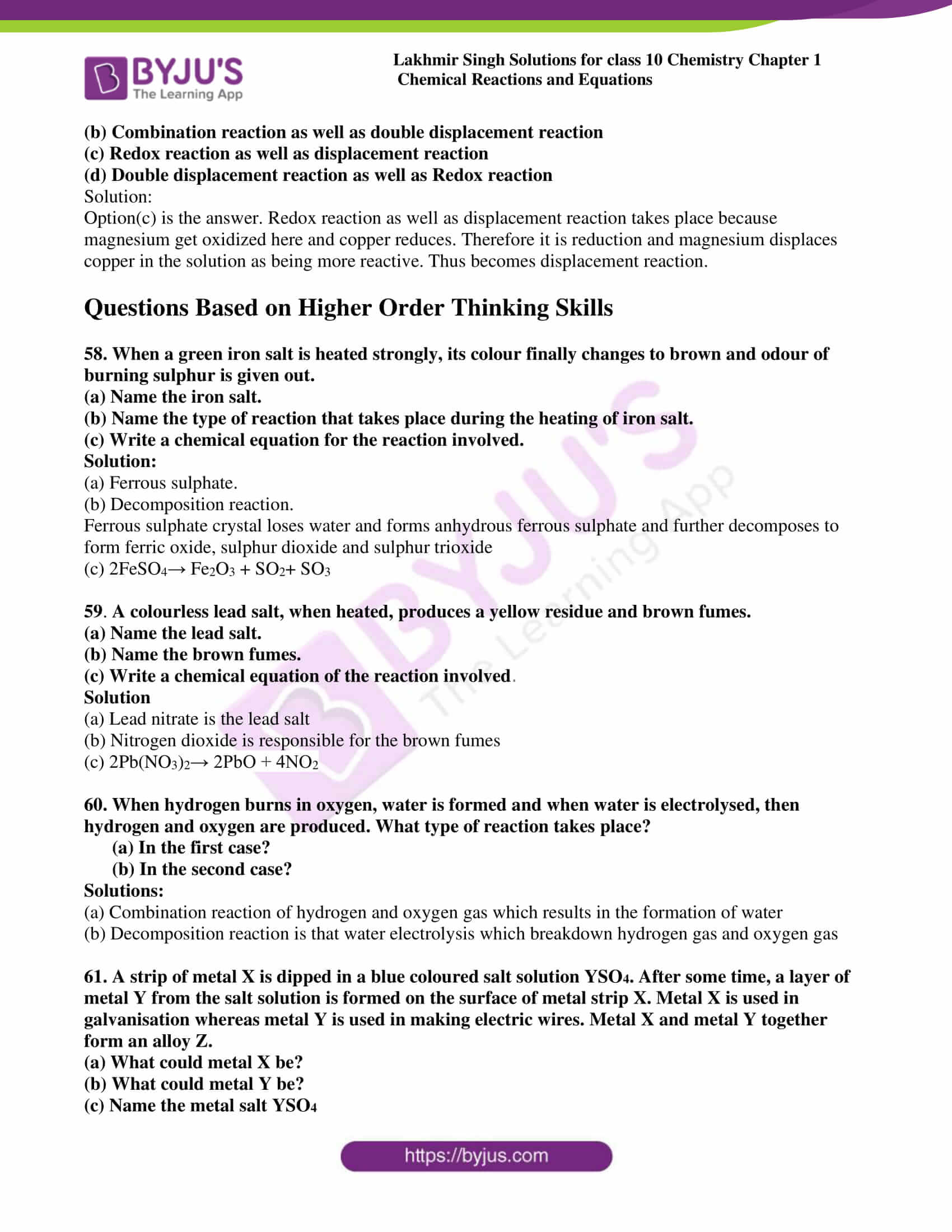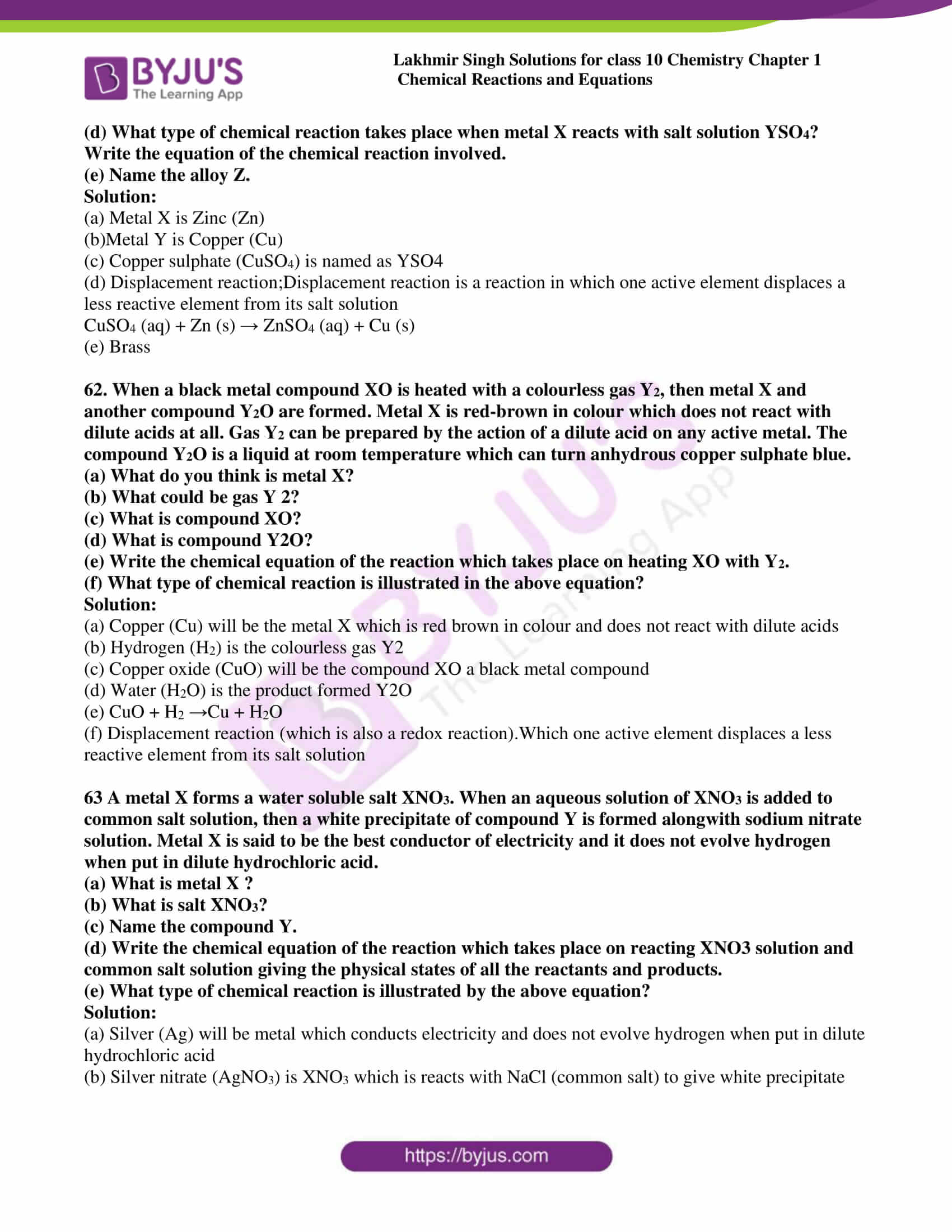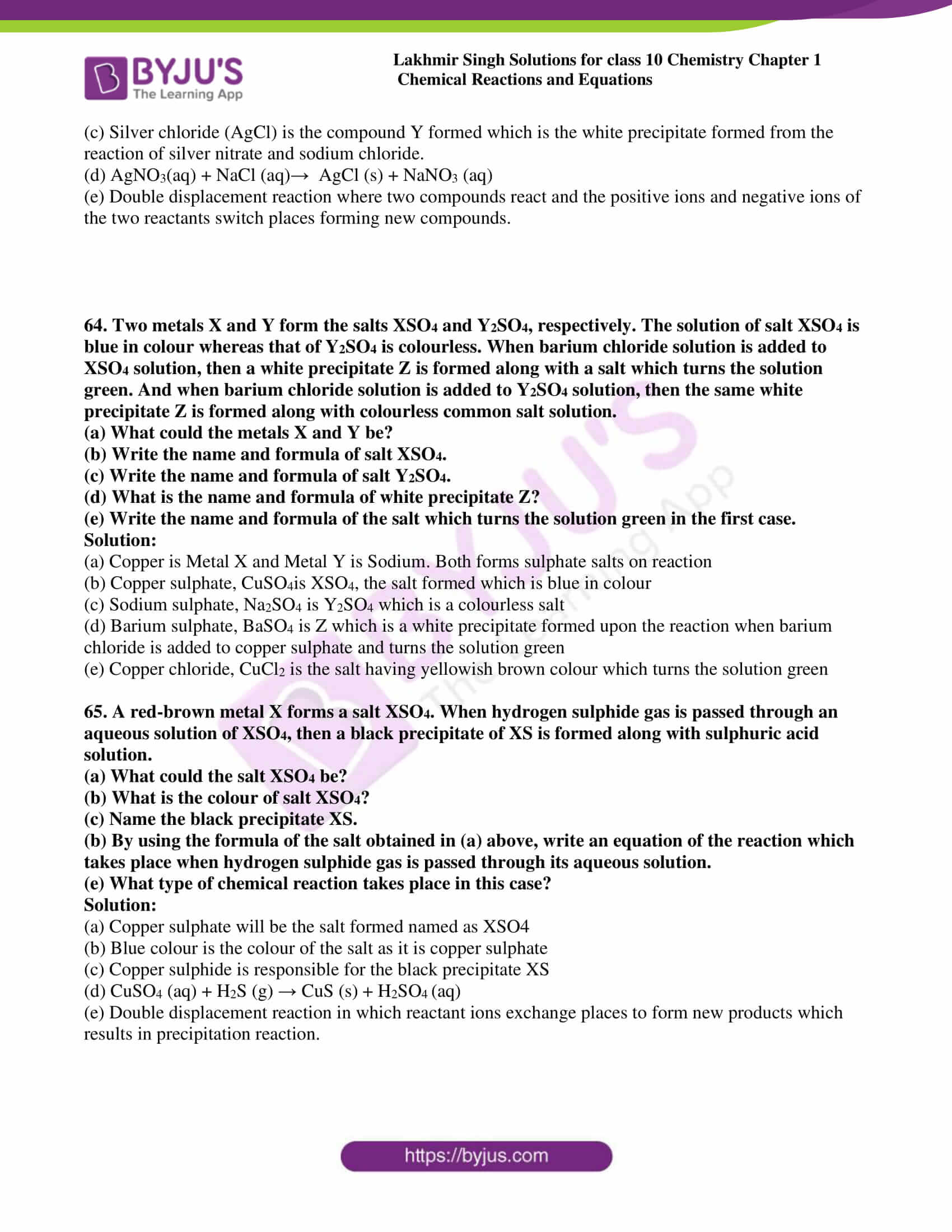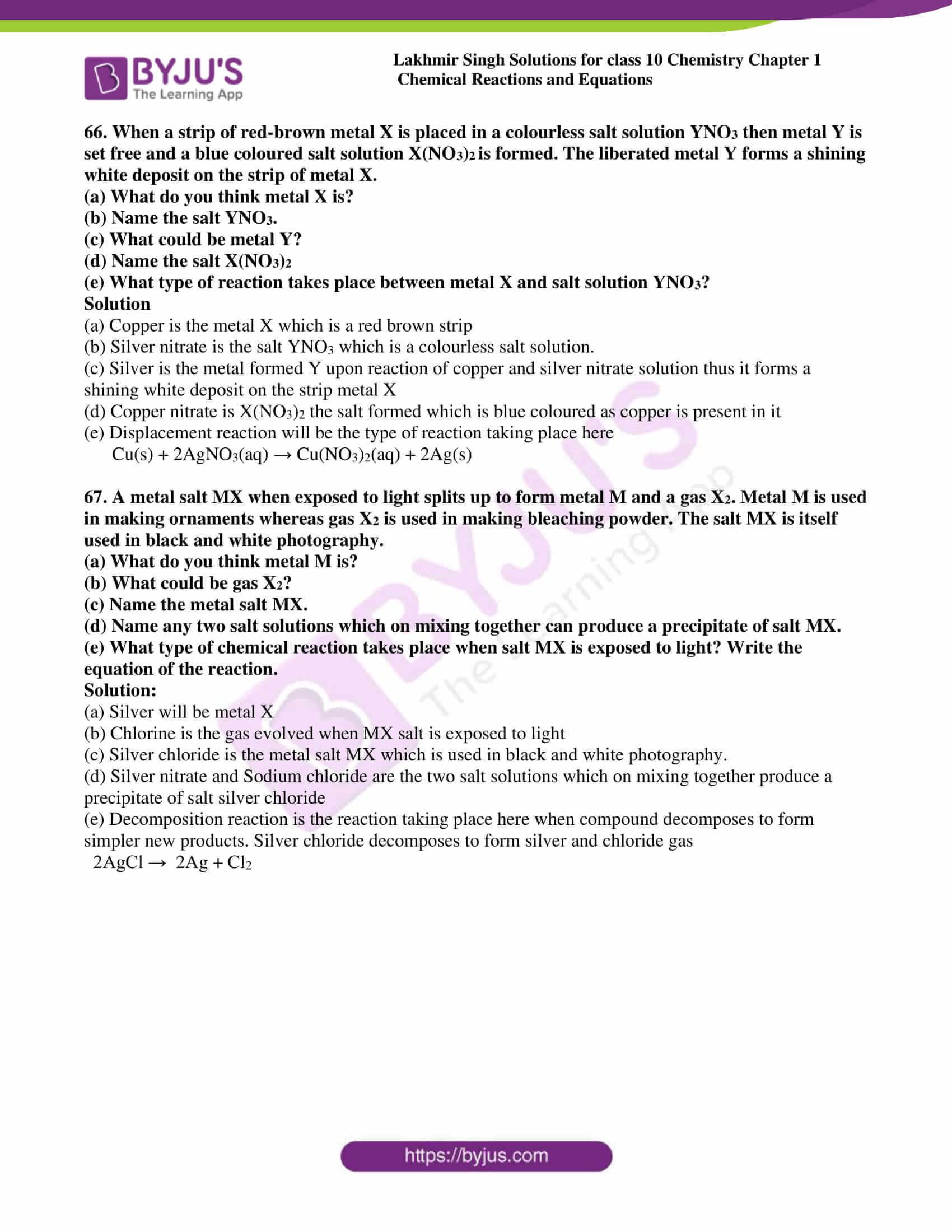### Access Lakhmir Singh Solutions For Class 10 Chemistry Chapter 1

Page no: 18

In-text questions set 1

1. Why is respiration considered as an exothermic process?

Solution:

Exothermic reaction is a reaction in which the energy is liberated. In the process of respiration, energy is released thus considered as an exothermic reaction

2. On what basis is a chemical equation balanced?

Solution:

A chemical equation is always balanced by comparing the number of atoms of each element on reactant side as well as product side. It is based on the Law of conservation of mass, i.e. number of atoms on the left hand side should be equal to the right hand side

3.What happens chemically when quicklime is added to water filled in a bucket?

Solution:

Quick lime is chemically calcium oxide. When it is added to water filled in a bucket, it forms calcium hydroxide (slaked lime) and reacts vigorously by releasing large amount of heat which is an exothermic reaction.

4.Why should magnesium ribbon be cleaned before burning in air?

Solution:

Magnesium ribbon is made up of pure magnesium metal and it is highly reactive with oxygen which further leads to the oxidation of magnesium when it comes in contact with air. On the surface of the magnesium ribbon it forms a layer of oxide and act as a protective layer. So it is necessary to clean the magnesium ribbon before burning in air

5. State whether the following statement is true or false:

A chemical equation can be balanced easily by altering the formula of a reactant or product.

Solution:

False

6. What is wrong with the following chemical equation?

Mg + O  MgO

Correct and balance it.

Solution:

Oxygen should be in molecular form, O2

2Mg + O2 → 2MgO

7.What does the symbol (aq) represent in a chemical equation?

Solution:

In a chemical equation (aq) always represents for aqueous solution

8.Why is photosynthesis considered an endothermic reaction?

Solution:

Endothermic reactions are those which heat is absorbed in the reaction. As we know that photosynthesis is a process that green plants and some other organisms use sunlight to synthesize food from carbon dioxide and water and turns to oxygen and glucose. Plants absorb direct energy from sun thus considered photosynthesis as endothermic reaction

9.How will you indicate the following effects in a chemical equation?

(a) A solution made in water

(b) Exothermic reaction

(c) Endothermic reaction

Solution:

(a) The solution made in water can be indicated by the symbol (aq) which shows it is aqueous

(b) An exothermic reaction can be indicated by Heat, heat energy or energy on the product side

(c) An endothermic reaction can be indicated by Heat, heat energy or energy on the reactant side

10. Translate the following statements into chemical equations and then balance the equations:

(a)   Hydrogen sulphide gas burns in air to give water and sulphur dioxide.

(b)   Phosphorus burns in oxygen to give phosphorus pentoxide.

(c)   Carbon disulphate burns in air to give carbon dioxide and sulphur dioxide.

(d)   Aluminium metal replaces iron from ferric oxide, Fe2O3, giving aluminium oxide and iron.

(e)  Barium chloride reacts with zinc sulphate to give zinc chloride and barium sulphate.

Solution:

(a) 2H2S + 3O2 → 2H2O + 2SO2

(b) P4 + 5O2 → 2P2O5

(c) CS+ 3O2 → CO2 + 2SO2

(d) 2Al + Fe2O3 → Al2O3 + 2Fe

(e) BaCl2 + ZnSO4 → ZnCl2 + BaSO4

11. Write the balanced chemical equations for the following reactions:

(a) Calcium hydroxide + Carbon dioxide  Calcium carbonate + Water

(b) Aluminium + Copper chloride  Aluminium chloride + Copper

Solution:

(a) Ca(OH)2 + CO2 → CaCO3 + H2O

(b) 2Al + 3CuCl→ 2AlCl3 + 3Cu

Page no: 19

12.Complete and balance the following equations:

(a) NaOH + ______  Na2SO4 + H2O

(b) Ca(OH)2 + _______  CaCO3 + H2O

Solutions:

(a) 2NaOH + H2SO4 → Na2SO4 + 2H2O

(b) Ca(OH)2 + CO2 → CaCO3 + H2O

13.Correct and balance the following equations:

(i) Ca + H2 CaOH + H

(ii) N + H  NH3

Solutions:

(i) Ca + 2H2O → Ca(OH)2 + H2

(ii) N2 + 3H2 →2NH3

14. Write complete balanced equations for the following reactions:

(a) Calcium (solid) + Water (liquid) Calcium hydroxide (solution) + Hydrogen (gas)

(b) Sulphur dioxide (gas) + Oxygen (gas)  Sulphur trioxide (gas)

Solutions:

(a) Ca (s) + 2H2O (l) → Ca(OH)2 (aq) + H2 (g)

(b) 2SO2 (g) + O2 (g) → 2SO3 (g)

15.Balance the following equations:

(i) Na + O2 → Na2O

(ii) H2O2→ H2O + O2

(iii) Mg(OH)2 + HCl → MgCl2 +H2O

(iv) Fe +O2 → Fe2O3

(v) Al(OH)3 → Al2O3 +H2O

(vi) NH3 + CuO → Cu +N2 +H2O

(vii) Al2(SO4)3 + NaOH → Al(OH)3 + Na2SO4

(viii) HNO3 +Ca(OH)2 → Ca(NO3)2

(ix) NaOH +H2SO4 → Na2SO4 +H2O

(x) BaCl2 + H2SO4 → BaSO4 +HCl

Solutions:

(i) 4Na + O2  2Na2O

(ii) 2H2O2  2H2O + O2

(iii) Mg(OH)2 + 2HCl  MgCl2 + 2H2O.

(iv) 4Fe + 3O2  2Fe2O3

(v) 2Al(OH)3  Al2O3 + 3H2O

(vi) 2NH3 + 3CuO  3Cu + N2 + 3H2O

(vii) Al2(SO4)3 + 6NaOH  2Al(OH)3 + 3Na2SO4

(viii) 2HNO3 + Ca(OH)2 Ca(NO3)2 + 2H2O

(ix) 2NaOH + H2SO4  Na2SO4 + 2H2O

(x) BaCl2 + H2SO4  BaSO4 + 2HCl

16.Fill in the following blanks with suitable words:

(a) Chemical equations are balanced to satisfy the law of _______.

(b) A solution made in water is known as an ____ solution and indicated by the symbol _______.

Solutions:

(a) Conservation of mass

(b) Aqueous; (aq)

17. (a) Give one example of a chemical reaction.

(b) State two characteristics of the chemical reaction which takes place when dilute sulphuric acid is poured over zinc granules.

(c) Give two characteristics of the chemical reaction which occurs on adding potassium iodide solution to lead nitrate solution.

Solutions:

(a)A chemical reaction results in new substances by undergoing certain reactions and rearrangements. There will be reactants on the left hand side and products on the right hand side. An example for chemical reaction is

An example of combustion is methane + oxygen forms carbon dioxide and water. This can be written as a balanced symbol equation:

CH4 + 2O2  CO2 + 2H2O

(b) When dilute sulphuric acid is poured over zinc granules heat will liberate thus the reaction becomes exothermic

Hydrogen gas is evolved which burns with a sound

(c) 2KI (aq) + Pb(NO ) (aq) → PbI (↓) + 2 KNO (aq)

When solutions of lead nitrate and potassium iodide are mixed together a yellow precipitate of lead iodide is formed. This is an example for Double Displacement Reaction.

18. (a) What is a chemical equation? Explain with the help of an example.

(b) Giving examples, state the difference between balanced and unbalanced chemical equations.

(c) Balance the following chemical equations:

(i) NH3 → N2 + H2

(ii) C +CO2→ CO

Solutions:

(a) Chemical equations are the symbolic representation of chemical reactions in which the reactants and products are expressed in terms of chemical formulae. Reactant entities are given on the left side and product entities are given on the right side of a chemical equation

Example: Zn + H2SO4ZnSO4 + H2

(b) A balanced chemical equation is always energy and mass conservative whereas unbalanced chemical equation is neither energy nor mass conservative. A chemical equation can be written by balancing total number of atoms of each element in reactants and products while in unbalanced chemical equations the total number of atoms of each element in reactants and products are not equal

Example: KClO3KCl + 3O2 Unbalanced equation

2KCl032KCl + 3O2 Balanced equation

4.4

(c) (i) 2NH3 → N2 + 3H2

(ii) C +CO2→ 2CO

19.When hydrogen is passed over copper oxide, copper and steam are formed. Write a balanced equation for this reaction and state which of the chemicals are:

(i) Elements

(ii) Compounds

(iii) Reactants

(iv) Products

(v) Metals

(vi) Non-metals

Solutions:

H2 + CuO→  Cu + H2O

(i) Elements : H2 and Cu

(ii) Compounds : CuO and H2O

(iii) Reactants: H2 and CuO

(iv) Products: Cu and H2O

(v) Metal: Cu

(vi) Non-metal: H2

(b) Write balanced chemical equation from the following information:

An aqueous calcium hydroxide solution (lime water) reacts with carbon dioxide gas to produce a solid calcium carbonate precipitate and water.

Solutions:

(a)Ca(OH)2 (aq)+ CO2 (g)—3000°C CaCO3(s)+H2O(l)

A chemical equation can be informative by

1) The chemical states is indicated by (s) for solids, (l) for liquids and (g) for gases and (aq) for aqueous solution

2) Temperature written on the above of arrow

3) Arrow upwards shows compound or any gas formed escaped or liberated

4)Arrow downwards shows precipitated

5) Heat is written on reactant side to indicate exothermic and when on written product side it will be endothermic

b) Ca(OH)2 (aq) + CO2 (g)  CaCO3 (s) + H2O (l)

21. a) What is a balanced chemical equation? Why should chemical equations be balanced?

b) Aluminium burns in chlorine to form aluminium chloride (AlCl3). Write a balanced chemical equation for this reaction.

c) Potassium metal reacts with water to give potassium hydroxide and hydrogen gas. Write a balanced chemical equation for this reaction.

Solution:

(a) A chemical equation can be written by balancing total number of atoms of each element in reactants and products. A chemical equation should be balanced so that it can follow Law of conservation of mass.

(b) 2Al + 3Cl2  2AlCl3

(c) 2K + 2H2 2KOH + H2

Page no: 20

22.(a) Explain, with example, how the physical states of the reactants and products can be shown in a chemical equation.

(b) Balance the following equation and add state symbols:

Zn + HCl → ZnCl2 + H2

(c) Convey the following information in the form of a balanced chemical equation:

“An aqueous solution of ferrous sulphate reacts with an aqueous solution of sodium hydroxide to form a precipitate of ferrous hydroxide and sodium sulphate remains in solution.”

Solution:

(a) The physical state of the reactants and products in a chemical reaction can be shown by using the letters in the round brackets if it is solid then (s) liquid (l) gas (g) and aqueous (aq)

Example: Ca(OH)2 (aq) + CO2 (g)  CaCO3 (s) + H2O (l)

(b) Zn (s) + 2HCl (aq)  ZnCl2 (aq) + H(g)

(c)  FeSO4(aq) + 2NaOH (aq)  Fe(OH)2 (s) + Na2SO4 (aq)

23.Write any two observations in an activity which may suggest that a chemical reaction has taken place. Give an example in support of your answer

Solution:

(i) Change in temperature

When calcium oxide reacts vigorously with water to produce calcium hydroxide a large amount of heat is evolved and thus temperature of the system increases hence it confirms that a chemical reaction has been taken place.

(ii) Gas evolution

When calcium carbonate is heated it decomposes into calcium oxide and carbon dioxide. Here carbon dioxide is a gas and thus confirms a chemical reaction

24. (a) Aluminium hydroxide reacts with sulphuric acid to form aluminium sulphate and water. Write a balanced equation for this reaction.

(b) Balance the following chemical equation:

MnO2 + HCl → MnCl2 + Cl2 + H2O

Solution:

(a) 2Al(OH)3 + 3H2SO4  Al2(SO4)3 + 6H2O

(b) MnO2 + 4HCl  MnCl2 + Cl2 + 2H2O

25. Write the balanced chemical equations for the following reactions, and add the state symbols:

(a) Magnesium carbonate reacts with hydrochloric acid to produce magnesium chloride, carbon dioxide and water.

(b) Sodium hydroxide reacts with sulphuric acid to produce sodium sulphate and water.

Solution:

(a) MgCO3 (s) + 2HCl (aq) MgCl(aq) + CO2 (g) + H2O (l)

(b) 2NaOH (aq) + H2SO4 (aq) Na2SO4 (aq) + 2H2O (l)

26.Carbon monoxide reacts with hydrogen under certain conditions to form methanol (CH3OH). Write a balanced chemical equation for this reaction indicating the physical states of reactants and product as well as the conditions under which this reaction takes place.

Solution:

CO(g) + 2H2(g) → CH3OH

Pressure: 300atm

Temperature: 300℃

Catalyst: zinc oxide and chromium oxide

27.(a) Potassium chlorate (KClO3) on heating forms potassium chloride and oxygen. Write a balanced equation for this reaction and indicate the evolution of gas. (b) Rewrite the following information in the form of a balanced chemical equation:

Magnesium burns in carbon dioxide to form magnesium oxide and carbon.

Solution:

(a) 2KClO3 (s)  2KCl (s) + 3O(g)

(b) 2Mg + CO2  2MgO + C

28. (a) Substitute formulae for names and balance the following equations:

Calcium carbonate reacts with hydrochloric acid to produce calcium chloride, water and carbon dioxide gas.

(b) Write balanced chemical equation with state symbols for the following reaction:

Sodium hydroxide solution reacts with hydrochloric acid solution to produce sodium chloride solution and water.

Solutions:

(a) CaCO3 + 2HCl  CaCl2 + H2O + CO2

(b) NaOH (aq) + HCl (aq)  NaCl (aq) + H2O (l)

29.Ammonia reacts with oxygen to form nitrogen and water. Write a balanced chemical equation for this reaction. Add the state symbols for all the reactants and products.

Solution:

4NH3 (g) + 3O2 (g)  2N2 (g) + 6H2O (l)

30. Write a balanced chemical equation for the process of photosynthesis giving the physical states of all the substances involved and the conditions of the reaction.

Solution:

6CO2 (g) + 6H2O (l) → C6H12O6 (aq.) + 6O2 (g) in the presence of sunlight

31.Translate the following statement into chemical equation and then balance it:

‘Barium chloride solution reacts with aluminium sulphate solution to form a precipitate of barium sulphate and aluminium chloride solution’.

Solution:

3BaCl2 (aq) + Al2(SO4)3 (aq)  3BaSO4 (s) + 2AlCl3 (aq)

32.When potassium nitrate is heated, it decomposes into potassium nitrite and oxygen. Write a balanced equation for this reaction and add the state symbols of the reactants and products.

Solution:

2KNO3 (s) 2KNO2 (s) + O2 (g)

33.(a) What is meant by a chemical reaction? Explain with the help of an example.

(b) Give one example each of a chemical reaction characterised by:

(i) Evolution of a gas

(ii) Change in colour

(iii) Formation of a precipitate

(iv) Change in temperature

(v) Change in state.

Solutions:

(a) A chemical reaction results in new substances by undergoing certain reactions and rearrangements. There will be reactants on the left hand side and products on the right hand side.

An example of combustion is methane + oxygen forms carbon dioxide and water. This can be written as a balanced symbol equation:

CH4 + 2O2  CO2 + 2H2O

(b) (i) Evolution of gas

Reaction of Zinc and Hydrochloric acid

Zn + 2HCl → ZnCl2 + H2(g)

Hydrogen gas is released in the form of diatomic molecule

(ii) Change in colour

Cu(s) + O2(g) + H2O → Cu(OH)2(s) + O2(g)

Copper is in reddish brown colour and when reacts with oxygen and water it forms hydrated copper carbonate which is green in colour

(iii) Formation of precipitate

AgNO3(aq) + KCl(aq) →AgCl(s) + KNO3

When silver nitrate and potassium chloride in its aqueous form reacts a precipitate of potassium nitrate is obtained

(iv) Change in temperature

CaO + H20 →Ca(OH)2

When Quick lime is added to water to form slaked lime a change in temperature is obtained as it reacts vigorously and produce heat which is an exothermic reaction

(v) Change in state

NH3(g) + HCl(g) → NH4Cl(s)

Ammonia and Hydrogen chloride gas reacts to form Ammonium chloride which is a solid

Page no: 21

34.(a) State the various characteristics of chemical reactions.

(b) State one characteristics each of the chemical reaction which takes place when:

(i) Dilute hydrochloric acid is added to sodium carbonate

(iii) Dilute sulphuric acid is added to barium chloride solution

(iv) Quicklime is treated with water

(v) Wax is burned in the form of a candle

Solution:

(a) A chemical reaction is a reaction that takes place to form new substance by reacting with reactants. It is characterised by Evolution of a gas, Change in colour, Formation of a precipitate, Change in temperature, Change in state.

(b) (i) Evolution of Carbon dioxide gas

(ii) Change in colour from purple to colourless

(iii) Formation of a white precipitate of Barium sulphate

(iv) Change in temperature, an exothermic reaction

(v) Change in state from solid to liquid and gas

35.(a) What do you understand by exothermic and endothermic reactions?

(b) Give one example of an exothermic reaction and one of an endothermic reaction.

(c) Which of the following are endothermic reactions and which are exothermic reactions?

(i) Burning of natural gas

(ii) Photosynthesis

(iii) Electrolysis of water

(iv) Respiration

(v) Decomposition of calcium carbonate

Solution:

(a)Exothermic reaction is a reaction takes place when energy is released from the system into the surroundings

Endothermic reaction is a reaction takes place when energy is absorbed from surroundings in the form of heat

(b) The thermal decomposition of Limestone

CaCO3(s)→CaO (s)+CO2(g) This is an example for endothermic reaction

Burning of fuel is an example for exothermic reaction

C8H18(l)+25O2(g)→16CO2(g)+18H2O (g)+heat

(c) (i) and (iv) are exothermic reaction. (ii),(iii),(v) are endothermic reactions

Multiple choice questions (MCQ’s)

36. One of the following does not happen during a chemical reaction This is :

(a) Breaking of old chemical bonds and formation of new chemical bonds

(b) Formation of new substances with entirely different properties

(c) Atoms of one element change into those of another element to form new products

(d) A rearrangement of atoms takes place to form new products

Solution:

Option (c) is the answer. Atoms of one element does not change into another element to form products but the bonds between these atom will break and form products and atoms are rearrange also

37. Which one of the following does not involve a chemical reaction?

(a) Digestion of food in our body

(b) Process of respiration

(c) Burning of candle wax when heated

(d) Melting of candle wax on heating

Solution:

Option (d) is the answer. Wax changes its physical state on heating but not the properties so it does not involve any chemical change but involves physical change

38. You are given the solution of lead nitrate. In order to obtain a yellow precipitate you should mix it with a solution of

(a) Potassium chloride

(b) Potassium nitride

(c) Potassium sulphide

(d) Potassium iodide

Solution:

Option (d) is the answer. When a solution of lead nitrate reacts with potassium iodide it gives yellow precipitate of lead iodide

39. An acid which can decolourise purple coloured potassium permanganate solution is :

(a) Sulphuric acid

(b) Citric acid

(d) Carbonic acid

(d) Hydrochloric acid

Solution:

Option (b) is the answer. Reaction of purple-coloured potassium permanganate with citric acid is characterised by change in colour from purple to colourless

40. The chemical reaction between the two substances is characterised by a change in colour from orange to green. These two substances are most likely to be:

(a) Potassium dichromate solution and sulphur dioxide

(b) Potassium permanganate solution and sulphur dioxide

(c) Potassium permanganate solution and lemon juice

(d) Potassium dichromate solution and carbon dioxide

Solution:

Option (a) is the answer. Reaction between potassium dichromate and sulphur dioxide is characterised by change in colour. The orange colour of acidified potassium dichromate will change into green by passing sulphur dioxide

41. The chemical reaction between quicklime and water is characterised by:

(a) Evolution of hydrogen gas

(b) Formation of slaked lime precipitate

(c) Change in temperature of mixture

(d) Change in colour of the product

Solution:

Option (c) is the answer. Reaction of quicklime and water releases higher amount of energy and thus it is an exothermic reaction.

42. One of the following is an endothermic reaction. This is:

(a) Combination of carbon and oxygen to form carbon monoxide

(b) Combination of nitrogen and oxygen to form nitrogen monoxide

(c) Combination of glucose and oxygen to form carbon dioxide and water

(d) Combination of zinc and hydrochloric acid to form zinc chloride and hydrogen

Solution:

Option (b) is the answer. When nitrogen and oxygen are heated at very high temperature they form nitrogen monoxide and it is a endothermic reaction.

43. Which of the following is not an endothermic reaction ?

(a) CaCO3→ CaO + CO2

(b) 2H2O → 2H2 + O2

(c) 6CO2 + 6H2O → C6H12O6 + 6O2

(d) C6H12O6 + 6O2→ 6CO2 + 6H2O

Solution:

Option (d) is the answer. This is responsible for the respiration reaction in which glucose burns in oxygen to produce heat energy and thus an exothermic reaction

44. One of the following is an exothermic reaction. This is:

(a) Electrolysis of water

(b) Conversion of limestone into quicklime

(c) Process of respiration

(d) Process of photosynthesis

Solution:

Option (c) is the answer. Respiration is an exothermic reaction as the heat energy is released which maintains our body temperature.

45. The chemical equations are balanced to satisfy one of the following laws in chemical reactions. This law is known as:

(a) Law of conservation of momentum

(b) Law of conservation of mass

(c) Law of conservation of motion

(d) Law of conservation of magnetism

Solution:

Option (b) is the answer. Equations are balanced when the mass of atoms of different element in reactants side equal to the products side.

Questions Based on Higher Order Thinking Skills (HOTS)

46.When the solution of substance X is added to a solution of potassium iodide, then a yellow solid separates out from the solution.

(a) What do you think substance X is likely to be?

(b) Name the substance which the yellow solid consists of.

(c) Which characteristic of chemical reactions is illustrated by this example?

(d) Write a balanced chemical equation for the reaction which takes place.

Mention the physical states of all the reactants and products involved in the chemical equation.

Solution:

(c) Formation of precipitate

(d)Pb(NO3)(aq) + 2KI (aq) → PbI(s) + 2KNO(aq)

47. When water is added gradually to a white solid X, a hissing sound and a lot of heat is produced forming a product Y. A suspension of Y in water is applied to the walls of a house during white washing. A clear solution of Y is also used for testing carbon dioxide gas in the laboratory.

(a) What could be solid X? Write its chemical formula.

(b) What could be product Y? Write its chemical formula.

(c) What is the common name of the solution of Y which is used for testing carbon dioxide gas?

(d) Write chemical equation of the reaction which takes place on adding water to solid X.

(e) Which characteristics of chemical reactions are illustrated by this example?

Solution:

(a) Calcium oxide, CaO.

(b) Calcium hydroxide, Ca(OH)2

(c) Lime water.

(d) CaO + H2O →  Ca(OH)2

(e) Change in temperature.

48. When metal X is treated with a dilute acid Y, then a gas Z is evolved which burns readily by making a little explosion.

(a) Name any two metals which can behave like metal X.

(b) Name any two acids which can behave like acid Y.

(c) Name the gas Z.

(d) Is the gas Z lighter than or heavier than air?

(e) Is the reaction between metal X and dilute acid Y exothermic or endothermic?

(f) By taking a specific example of metal X and dilute acid Y,

Write a balanced chemical equation for the reaction which takes place.

Also indicate physical states of all the reactants and products.

Solution:

(a) Zinc and Iron.

(b) Dilute hydrochloric acid and dilute sulphuric acid.

(c) Hydrogen.

(d) Lighter than air.

(e) Exothermic.

(f) Suppose metal X is zinc (Zn) and acid Y is dilute hydrochloric acid (HCl) ;

Zn (s) + 2HCl (aq) → ZnCl2 (aq) + H2 (g)

49.A solid substance P which is very hard is used in the construction of many buildings, especially flooring. When substance P is heated strongly, it decomposes to form another solid Q and a gas R is given out. Solid Q reacts with water with the release of a lot of heat to form a substance S. When gas R is passed into a clear solution of substance S, then a white precipitate of substance T is formed. The substance T has the same chemical composition as starting substance P.

(a) What is substance P? Write its common name as well as chemical formula.

(b) What is substance Q?

(c) What is gas R?

(d) What is substance S? what is its clear solution known as?

(e) What is substance T? Name any two natural forms in which substance T occurs in nature.

Solution:

(a) Calcium carbonate (limestone), CaCO3

(b) Calcium oxide, CaO

(c) Carbon dioxide, CO2

(d) Calcium hydroxide, Ca(OH)2; Lime water.

(e) Calcium carbonate; Limestone and Marble. Page no:23

50. A silvery-white metal X taken in the form of ribbon, when ignited, burns in air with a dazzling white flame to form a white powder Y. When water is added to powder Y, it dissolves partially to form another substance Z.

(a) What could metal X be?

(b) What is powder Y?

(c) With which substance metal X combines to form powder Y?

(d) What is substance Z? Name one domestic use of substance Z.

(e) Write a balanced chemical equation of the reaction which takes place

When metal X burns in air to form powder Y.

Solution:

(a) Magnesium, Mg.

(b) Magnesium oxide, MgO

(c) Oxygen (of air), O2

(d) Magnesium hydroxide, Mg(OH)2; Used as antacid to relieve indigestion

(e) 2Mg + O2→ 2MgO

51. A metal X forms a salt XSO4. The salt XSO4 forms a clear solution in water which reacts with sodium hydroxide solution to form a blue precipitate Y. Metal X is used in making electric wires and alloys like brass.

(a) What do you think metal X could be?

(b) Write the name, formula and colour of salt XSO4.

(c) What is the blue precipitate Y?

(d) Write a chemical equation of the reaction which takes place

When salt XSO4 reacts with sodium hydroxide solution.

Give the state symbols of all the reactants and products which occur in the above equation.

Solution:

(a) Metal could be Copper, Cu.

(b) Copper sulphate, CuSO4, Blue colour.

(c) The blue precipitate Y is Copper hydroxide, Cu(OH)2

(d) CuSO4 (aq) + 2NaOH (aq) → Cu(OH)2 (s) + Na2S04 (aq)

52. The metal M reacts vigorously with water to form a solution S and a gas G. The solution S turns red litmus to blue whereas gas G, which is lighter than air, burns with a pop sound. Metal M has a low melting point and it is used as a coolant in nuclear reactors.

(a) What is metal M?

(b) What is solution S? Is it acidic or alkaline?

(c) What is gas G?

(d) Write a balanced chemical equation for the reaction which takes place when metal M reacts with water.

(e) Is this reaction exothermic or endothermic?

Solution:

(a) Sodium is the metal ( Na )

(b) Solution S is Sodium hydroxide solution (NaOH solution),It is alkaline.

(c) Hydrogen, H2is the gas evolved

(d) 2Na + 2H2O → 2NaOH + H2

(e) Reaction is exothermic.

53.When a mixture of gases X and Y is compressed to 300 atm pressure and then passed over a catalyst consisting of a mixture of zinc oxide and chromium oxide (heated to a temperature of 300oC), then an organic compound Z having the molecular formula CH4O is formed. X is a highly poisonous gas which is formed in appreciable amounts when a fuel burns in a limited supply of air; Y is a gas which can be made by the action of a dilute acid on an active metal; and Z is a liquid organic compound which can react with sodium metal to produce hydrogen gas.

(a) What are X, Y and Z?

(b) Write a balance chemical equation of the reaction which takes place

When X and Y combine to form Z. Indicate the conditions under which the reaction occurs.

Solution:

(a) X= Carbon monoxide gas (CO); Y= Hydrogen gas (H2); Z = Methanol (CH3OH or CH4O)

(b)CO(g) + 2H2(g) → CH3OH (l)

Carbon monoxide when reacts with hydrogen gas under a pressure of 300atmosphere and a temperature of 300℃ and a catalyst which is a mixture of Zinc oxide and chromium oxide

54.The white solid compound A decomposes quite rapidly on heating in the presence of a black substance X to form a solid compound B and a gas C. When an aqueous solution of compound B is reacted with silver nitrate solution, then a white precipitate of silver chloride is obtained alongwith potassium nitrate solution. Gas C does not burn itself but helps burn things.

(a) What is compound A?

(b) What is compound B?

(c) What is gas C?

(d) What do you think is the black substance X? What is its function?

(e) What is the general name of substance like X?

Solution:

(a) Compound A is Potassium chlorate, KClO3

(b) Compound B is Potassium chloride, KCl

(c) Gas C is Oxygen, O2

(d) X is Manganese dioxide, MnO2; Manganese dioxide is used as a catalyst in order to enhance the reaction

(e) Catalysts are the general name for substances like X

55. Gas A, which is the major cause of global warming, combines with hydrogen oxide B in nature in the presence of an environmental factor C and a green material D to form a six carbon organic compound E and a gas F. The gas F is necessary for breathing.

(a) What is gas A?

(b) What is the common name B?

(c) What do you think could be C?

(d) What is material D? Where is it found?

(e) Name the organic compound E.

(f) What is gas F? Name the natural process during which it is released.

Solution:

(a) Gas A is Carbon dioxide, CO2

(b) B is Water, H2O

(c) Sunlight is the environmental factor

(d) Material D is Chlorophyll; it is found in green leaves of plants which provide pigmentation

(e) Organic compound E is Glucose, C6H12O6

(f) F is Oxygen; Photosynthesis is the process where Oxygen is released

Page no: 45

1. What type of reaction is represented by the digestion of food in our body?

Solution:

Decomposition reaction is the reaction which is represented by the digestion of our body it is nothing but a chemical reaction where food what we are having is further break down into digestible nutrients such as fats, proteins and carbohydrates which is essential for the proper functioning of a human body

2. Name the various types of chemical reactions.

Solutions:

There are many types of chemical reactions. Here are the names:

(i) Combination reactions.

(ii) Decomposition reaction.

(iii) Displacement reaction.

(iv) Double displacement reaction.

(v) Oxidation and reduction reactions.

3. Why does the colour of copper sulphate solution change when an iron nail is kept immersed in it?

Solutions:

It is a displacement reaction. Copper sulphate solution changes its colour because iron nail dipped in the solution is more reactive than copper sulphate and it displaces the molecules. The copper gets deposited over the nail as the copper sulphate solution becomes iron sulfate solution and colour changes from blue to green

4. Write the balanced chemical equation for the following reaction:

Zinc + Silver nitrate Zinc nitrate + Silver

Solution:

Zn + 2AgNO3 →  Zn(NO3)2 + 2Ag

5. Which term is used to indicate the development of unpleasant smell and taste in fat and oil containing foods due to aerial oxidation (when they are kept exposed for a considerable time)?

Solution:

Rancidity is the term used and this condition is produced by aerial oxidation of unsaturated fat present in food and other products. It is incomplete oxidation of fats and oils when exposed to air, light or moisture

6.What is the general name of the chemicals which are added to fat and oil containing foods to prevent the development of rancidity?

Solution:

Anti-oxidants are the chemicals which are added to fat and oil containing foods to slow or delay the development of rancidity. Natural Anti-oxidants are ascorbic acid ad tocopherols whereas synthetic anti-oxidants include Butylated hydroxyanisole (BHA) and Butylated hydroxytoluene (BHT)

7. State an important use of decomposition reactions.

Solution:

Decomposition of silver chloride into silver and chlorineby light is used in photography.

In the human body, this reaction helps in digestion of food in the stomach.

Decomposition reaction is used in the manufacture of cement and gypsum.

This reaction is used in the process of welding.

8. What are anti-oxidants? Why are they added to fat and oil containing foods?

Solution:

Anti-oxidants are the chemicals which are added to fat and oil containing foods to slow or delay the development of rancidity. Natural Anti-oxidants are ascorbic acid ad tocopherols whereas synthetic anti-oxidants include Butylated hydroxyanisole (BHA) and Butylated hydroxytoluene (BHT). Anti-oxidants can be nutrients as well as enzymes that are especially proteins that can assist in chemical reaction and prevent development such as cancer,heart attack and many other chronic diseases

9. Explain why, food products containing fats and oils (like potato chips) are packaged in nitrogen.

Solution:

The packet of chips or foods containing fats and oils are flushed with nitrogen. This is because nitrogen acts as a antioxidant and this packaging is to prevent the food products from being oxidized. If we pack with oxygen it reacts and become rancid and the smell and taste will change and will start to decay.

10. Give one example of a decomposition reaction which is carried out:

(a) With electricity

(b) By applying heat

Solution:

(a) When electricity is passed through acidified water it decomposes to Hydrogen and oxygen gas

2H2 O → 2H2 + O2

(b) By applying heat, thermal decomposition takes place

2NaHCO3(s) → CO2(g) + H2O(g) + Na2CO3(s)

Heating of baking soda is an example

11. What type of chemical reaction is used to extract metals from their naturally compounds like oxides or chlorides?

Solutions:

Decomposition reaction is used to extract several metals from their naturally occurring compounds like oxide and chloride

Example: 2NaCl → 2Na + Cl2

12. Name two anti-oxidants which are usually added to fat and oil containing foods to prevent rancidity.

Solution:

Anti-oxidants are used as preservatives in fat containing foods to slow up the development of rancidity due to oxidation

Butylated hydroxytoluene (BHT) and Butylated hydroxyanisole (BHA) can also be used as anti-oxidants with greater half-life than natural anti-oxidants.

13.Write one equation each for the decomposition reactions where energy is supplied in the form of (a) heat, (b) light, and (c) electricity.

Solution:

(a) Decomposition reaction when heat is supplied

2Pb(NO3)2→ 2PbO + 4NO2 + O2

(b) Decomposition reaction when light is supplied

2AgCl → 2Ag + Cl2

(c) Decomposition reaction where electricity is supplied

2H2O→ 2H2 + O2

14. In the refining of silver, the recovery of silver from silver nitrate solution involved displacement by copper metal. Write down the chemical equation of the reaction involved.

Solution:

2AgNO3 (aq) + Cu (s) → Cu(NO3)2 (aq) + 2Ag (s)

15. What type of reactions are represented by the following equations?

(i) CaCO3→  CaO + CO2

(ii) CaO + H2O →  Ca(OH)2

(iii) 2FeSO4→ Fe2O3 + SO2+ SO3

(iv) NH4Cl →  NH3 + HCl

(v) 2Ca + O2→  2CaO

Solution:

(i) Decomposition.

(ii) Combination.

(iii) Decomposition.

(iv) Decomposition.

(v) Combination.

Page no: 46

16.What type of chemical reaction stake place when:

(a) A magnesium wire is burnt in air?

(b) Lime-stone is heated?

(c) Silver bromide is exposed to sunlight?

(d) Electricity is passed through water?

(e) Ammonia and hydrogen chloride are mixed?

Solution:

(a) Combination.

(b) Decomposition.

(c) Decomposition.

(d) Decomposition.

(e) Combination.

17. What type of chemical reactions is represented by the following equations?

(i) A + BC → AC+ B

(ii) A +B → C

(iii) X → Y+ Z

(iv) PQ + RS → PS + RQ

(v) A2O3 + 2B → B2O3 + 2A

Solution:

(i) Displacement reaction.

(ii) Combination reaction.

(iii) Decomposition reaction.

(iv) Double displacement reaction.

(v) Displacement reaction.

18. Balance the following chemical equations:

(a) FeSO4→ Fe2O3 + SO2 + SO3

(b) Pb(NO3)2(s) →PbO(s) + NO2(g) + O2

Solutions:

(a) 2FeSO4→ Fe2O3 + SO2 + SO3

(b) 2Pb(NO3)2(s) → 2PbO(s) + 4 NO2(g) + O2

Both in the presence of heat and decomposition reaction takes place

19. Which of the following is a combination and which a displacement reaction.

(a) Cl2 +2KI → 2KCl +I2

(b) 2K + Cl2 → 2KCl

Solution:

(a) Displacement reaction.

(b) Combination reaction.

20.What type of reactions are represented by the following equations?

(a) CaO + CO2→ CaCO3

(b) 2Na +2H2O → 2NaOH +H2

(c) Mg + CuSO4→ MgSO4 + Cu

(d) NH4NO2→ N2 +2H2O

(e) CuSO4 + 2NaOH →Cu(OH)2 +Na2SO4

Solution:

(a) Combination reaction.

(b) Displacement reaction.

(c) Displacement reaction.

(d) Decomposition reaction.

(e) Double displacement reaction

21. In the following reaction between lead sulphide and hydrogen peroxide:

PbS(s) + 4H2O2(aq) → PbSO4(s) +4H2O(l)

(a) Which substance is reduced?

(b) Which substance is oxidized?

Solution:

(a) H2O2

(b) PbS

22. Identify the component oxidized in the following reaction:

H2S +Cl2→ S +2HCl

Solution:

H2S is oxidized in the above reaction.

23. When SO2 gas is passed through saturated solution of H2S, the following reaction occurs:

SO2 + 2H2S → 2H2O +3S

In this reaction, which substance is oxidized and which one is reduced?

Solution:

In this reaction the substance oxidised is H2S and the substance which got reduced is SO2

24. Fill in the following blanks with suitable words:

(a) The addition of oxygen to a substance is called ______ whereas removal of oxygen is called ______.

(b) The addition of hydrogen to a substance is called ______ whereas removal of hydrogen is called ______.

(c) Anti-oxidants are often added to fat containing foods to prevent ______ due to oxidation

Solution:

(a) Oxidation; reduction.

(b) Reduction; oxidation.

(c) Rancidity.

25. What is an oxidation reaction? Identify in the following reaction (i) the substance oxidized, and (ii) substance reduced:

ZnO + C → Zn +CO

Solution:

Oxidation is the process when an atom loses its electron in a reaction. Or the addition of oxygen to a substance to increase the proportion of oxygen in its molecule and oxidation can be achieved without oxygen by the removal of hydrogen i.e. dehydrogenation.

(i)Substance oxidized is C (ii) Substance reduced is ZnO

26. a) What is a redox reaction? Explain with an example.

(b) When a magnesium ribbon burns in air with a dazzling flame and forms a white ash, is magnesium oxidised or reduced? Why?

(c) In the reaction represented by the equation:

MnO2 + 4HCl → MnCl2 +2H2O +Cl2

(i) Name the substance oxidized           (ii) Name the oxidizing agent

(ii) Name the substance reduced           (iv) Name the reducing agent

Solutions:

(a)A redox reaction is a reaction where oxidation and reduction occurs simultaneously. It is also called redox reaction.

SO3(aq) + MnO4(aq) → SO4(aq) + Mn

Oxidation happened for SO3and reduction happened for MnO4

(b) Magnesium is oxidised as addition of oxygen to magnesium takes place leading to formation of magnesium oxide.

(c) (i) HCl (ii) MnO2(iii) MnO2 (iv) HCl

Page no: 47

27. (a) Define a combination reaction.

(b) Give one example of a combination reaction which is also exothermic.

(c) Give one example of a combination reaction which is also endothermic.

Solution:

(a) Combination reactions are those reactions in which two or more substances combine to form a single substance or product or two reactants combine to form one product

(b) C (s) + O2 (g) →CO2 + Heat (Burning of carbon in presence of oxygen to produce carbon dioxide)

(c) N2 (g) + O(g) + Heat → 2NO (g)

28.(a) Give an example of an endothermic reaction?

(b) Is oxidation an exothermic or an endothermic reaction?

(c) Explain, by giving an example, how oxidation and reduction processed side by side.

Solution:

(a) Endothermic reaction is a reaction heat is absorbed by the reaction and example is burning of carbon in presence of oxygen gives carbon dioxide and a large amount of heat is released

C (s) + O2 (g) →CO2 + Heat

(b) Oxidation is an exothermic reaction. Oxidation is areaction which oxygen combines with other elements and exothermic reaction releases energy through light or heat

(c) CuO + H2→ Cu + H2O

In this above reaction, Copper oxide is reduced to copper and hydrogen is oxidized to water

29. (a) What is the colour of ferrous sulphate crystals? How does this colour change after heating?

(b) Name the product formed on strongly heating ferrous sulphate crystals. What type of chemical reaction occurs in this change?

Solution:

(a)Ferrous sulphate crystals are green in colour and after heating it becomes white because on heating ferrous sulphate crystals loses water and anhydrous ferrous sulphate FeSO4 is formed so the colour changes from light green to white.

(b) On strong heating the anhydrous sulphate decomposes to form Ferric oxide, sulphur dioxide and sulphur trioxide. This is a decomposition reaction

2FeSO4→ Fe2O3 + SO2+ SO3

30. What is a decomposition reaction? Give an example of a decomposition reaction. Describe an activity to illustrate such a reaction by heating.

Solution:

Decomposition reaction is a reaction where the compounds break down or splits into two or more products. They are classified into three. Thermal decomposition, electrolytic decomposition and photo decomposition reaction

There are so many examples for decomposition reactions one of them is decomposition of calcium carbonate into calcium oxide and carbon dioxide when heated

Decomposition reaction by heating is called Thermal decomposition reaction. Here is an example for that kind of reaction

2KClO3(s) + Heat → 2KCl + 3O2

Potassium chromate decomposes into potassium chloride and oxygen gas is evolved.

31. Zinc oxide reacts with carbon, on heating, to form zinc metal and carbon monoxide. Write a balanced chemical equation for this reaction. Name (i) oxidizing agent, and (ii) reducing agent, in this reaction.

Solution:

ZnO + C → Zn + CO

(i) Oxidizing agents is a reactant which gain electrons and reduced during a redox reaction and here Zinc oxide is the oxidizing agent

(ii) Reducing agent or reductant is that which substance causes another substance to reduce or it loses electron and oxidized in a chemical reaction. Here carbon is reducing agent.

32.Give one example of an oxidation-reduction reaction which is also:

(a) A combination reaction

(b) A displacement reaction

Solution:

(a) 2Cu + O2 → 2CuO (Heat is given)

Copper combines with oxygen to form copper oxide and it is a oxidation-reduction reaction

(b) CuO + H2→ Cu + H2O

Here hydrogen displaces copper to form dihydrogen oxide (Water)

33.(a) What is the difference between displacement and double displacement reactions? Write equations for these reactions.

(b) What do you mean by a precipitation reaction? Explain giving an example.

Solution:

Displacement reaction is a chemical reaction in which a more reactive element displaces a less reactive element from its compound. Both metals and non-metals take part in displacement reaction.

Example for displacement reaction is

CuSO4 + Zn → ZnSO4+ Cu

Double displacement reaction takes place when two atoms or group of atoms switch places forms new compound. It takes place generally in aqueous solutions.

Example for double displacement reaction is

Na2SO4 + BaCl2 → BaSO4 + 2NaCl

(b) Precipitation reaction is a reaction which an insoluble solid called precipitate formed thatseparates from the solution.

Example is when barium chloride solution is added to sodium sulphate solution a white precipitate of barium sulphate is formed along with sodium chloride solution.

Na2SO4 + BaCl2 → BaSO4 + 2NaCl

34. (a) Explain the following in terms of gain or loss of Oxygen with one example each:

(i) Oxidation (ii) reduction

(b) When copper powder is heated strongly in air, it forms copper oxide. Write a balanced chemical equation for this reaction. Name (i) substance oxidised, and (ii) substance reduced

Solution:

(a) Oxidation is addition of oxygen and reduction is removing of oxygen

H2S + Cl2→ S + 2HCl

Here, hydrogen is removed from hydrogen sulphide so it is oxidation and hydrogen sulphide is oxidized to sulphur.

(b) 2Cu + O2 → 2Cu0

(i) Cu is oxidized (ii) O2is reduced

35. (a) Define the following in terms of gain or loss of hydrogen with one example each:

(i) Oxidation (ii) reduction

(b) When a magnesium ribbon is heated, it burns in air to form magnesium oxide. Write a balanced chemical equation for this reaction. Name (i) substance oxidised, and (ii) substance reduced.

Solution:

Oxidation is removal of hydrogen and reduction is gaining of hydrogen. By taking an example

Fe2O3 + 3CO → 2Fe + 3CO2

Fe got reduced here and Carbon got oxidized by gaining oxygen

(b) 2Mg + O2→ 2MgO

(i) Substance oxidized is Mg (ii) Substance reduced is O2

36. What is meant by (a) displacement reaction, and (b) double displacement reaction? Explain with the help of one example each.

(a) Displacement reaction is a reaction in which one active element displaces a less reactive element from its salt solution

2KI + Cl2 →  2KCl + I2

(b) Double displacement reaction is a chemical change in which two ionic compounds are exchanged and make components

Fe2O3 + 6HCl → 2FeCl3 + 3H2O

37. (a) Why are decomposition reactions called the opposite of combination reactions? Explain with equations of these reactions.

(b) Express the following facts in the form of a balanced chemical equation:

“When a strip of copper metal is placed in a solution of silver nitrate, metallic silver is precipitated and a solution containing copper nitrate is formed.”

Solution:

The reactions those split or break the compounds into two simpler products are called decomposition reaction

Example: decomposition of calcium carbonate into calcium oxide and carbon dioxide when heated

CaCO3→  CaO + CO2

They are called opposite of combination reaction because in combination reaction two or more reactants combined to form one single product

Example Magnesium and oxygen combine to form magnesium dioxide

2Mg + O2→ 2MgO

(b) Cu (s) + 2AgNO3 (aq)→  Cu(NO3)2 (aq) + 2Ag (s)

38. a) What happens when a piece of iron metal is placed in copper sulphate solution? Name the type of reaction involved.

(b) Write balanced chemical equation with state symbols for the following reaction:

Barium chloride solution reacts with sodium sulphate solution to give insoluble barium sulphate and a solution of sodium chloride.

Solution:

(a) When a piece of iron metal is placed in copper sulphate solution, then magnesium sulphate solution and copper metal are formed. This is a type of displacement reaction.

CuSO4 + Fe → FeSO4 + Cu

(b) Na2SO4 + BaCl2 → BaSO4 + 2NaCl

39.In the reaction represented by the following equation:

(a) Name the substance oxidised

(b) Name the substance reduced

(c) Name the oxidising agent

(d) Name the reducing agent

Solutions:

(a) H2 (Addition of oxygen dihydrogen)

(b) CuO (Removal of oxygen)

(c) CuO (Beingreduced)

(d) H2(Being oxidized)

40.What happens when silver nitrate solution is added to sodium chloride solution?

(a) Write the equation for the reaction which takes place.

(b) Name the type of reaction involved.

Solutions:

When silver nitrate solution is added to sodium chloride solution, a white precipitate of silver chloride is formed alongwith sodium nitrate solution.

(a)AgNO3 (aq) + NaCl (aq) → AgCl (s)+ NaNO3 (aq)

(b) Double displacement reaction that is a chemical reaction where two compounds react and the positive ions and negative ions of the two reactants switch places forming new compounds.

41. What happens when silver chloride is exposed to sunlight? Write a chemical equation for this reaction. Also give one use of such a reaction.

Solution:

When silver chloride is exposed to light, it decomposes to form silver metal and chlorine gas which is a photolytic decomposition reaction,decomposition carried out by sunlight.

2AgCl(s) → 2Ag(s) + Cl2

This reaction is used in black and white photography.

42.What happens when a zinc strip is dipped into a copper sulphate solution?

(a) Write the equation for the reaction that takes place.

(b) Name the type of reaction involved.

Solution:

Zinc sulphate Solution and copper are obtained when a strip of zinc metal is placed in copper Sulphate Solution.

(a) CuSO4(aq.) +Zn(s)→  ZnSO4(aq.) +Cu(s)

(b) Displacement reaction takes place where zinc metal displaces as zinc sulphate and copper sulphate to copper.

43.(a) Explain the term “corrosion” with an example. Write a chemical equation to show the process of corrosion of iron.

(b) What special name is given to the corrosion of iron?

(c) What type of chemical reaction is involved in the corrosion of iron?

(d) Name any three objects (or structures) which are gradually damaged by the corrosion of iron and steel.

Solutions:

Corrosion is a natural process that gradually destructs the materials by chemically or by electrochemical reaction within the environment. Corrosion occurs when most or all of the atoms on the same metal surface are oxidized and damaging the entire surface. Rusting of iron is the most common form of corrosion

4Fe + 3O2 + 2H2O → 2Fe2O3.xH2O

(b) Rusting

(c) Oxidation

(d) Statues, cars, buildings

44. (a) Explain the term “rancidity”. What damage is caused by rancidity?

(b) What type of chemical reaction is responsible for causing rancidity?

(c) State and explain the various methods for preventing or retarding rancidity of food.

Solution:

(a)Rancidity is the process of slow oxidation of oil and fat present in the food materials resulting in the production of foul odor and taste in them. When fats and oils are oxidized, the food becomes rancid i.e., their smell and taste changes.

(b)Oxidation is the chemical reaction involved in this as it get exposed to air

(c) (i) Refrigerate cooked food at low temperatures

(ii) Pack food items in air tight containers

(iii) Prevention by adding anti-oxidants like BHA(Butylated hydroxyanisole)

(iv) Storage of food away from light

45.(a) What happens when an aqueous solution of sodium sulphate reacts with an aqueous solution of barium chloride?

(b) Write the balanced chemical equation for the reaction which takes place.

(c) State the physical conditions of reactants in which the reaction will not take place.

(d) Name the type of chemical reaction which occurs.

(e) Give one example of another reaction which is of the same type as the above reaction.

Solution:

(a) When barium chloride solution is added to sodium sulphate solution, then a white precipitate of barium sulphate is formed alongwith sodium chloride solution.

Na2SO4 + BaCl2 → BaSO4 + 2NaCl

(c) Solid sodium sulphate and Solid barium chloride

(d) Double displacement reaction.

(e)Silver nitrate solution and sodium chloride solution forms a white precipitate of silver chloride and sodium nitrate solution.

Multiple Choice Questions(MCQ’S)

46. The removal of oxygen from a substance is called

(a) Oxidation

(b) Corrosion

(c) Reduction

(d) Rancidity

Solution:

Option (c) is correct. Reduction is the process of removal of oxygen from a substance and adding of hydrogen. It occurs when the oxidation of atoms decreases

47. In the context of redox reactions, the removal of hydrogen from a substance is known as :

(a) Oxidation

(b) Dehydration

(c) Reduction

(d) Dehydrogenation

Solution:

Option (a) is the answer. In redox reaction both reduction and oxidation takes place and reduction is when hydrogen is added and oxidation is where hydrogen is removed

48. The chemical reaction involved in the corrosion of iron metal is that of:

(a) Oxidation as well as displacement

(b) Reduction as well as combination

(c) Oxidation as well as combination

(d) Reduction as well as displacement

Solution:

Corrosion takes place when iron metal reacts with water and oxygen and form hydrated oxide and is an oxidation reaction as it is adding up of oxygen

Iron metal combines with water and oxygen to corrode thus it is a combination reaction which further forms a single compound.

49. The term used to indicate the development of unpleasant smell and taste in fat and oil containing food due to aerial oxidation is:

(a) Acidity

(c)Rabidity

(d)Rancidity

Solution:

Option (d) is the answer. Rancidity is the term used to indicate the development of unpleasant smell and taste in fat and oil containing food due to aerial oxidation and it can be prevented by closely and tight packing of food items in a container which does not allows air to react.

50. In order to prevent the spoilage of potato chips, they are packed in plastic bags in an atmosphere of:

(a) Cl2

(b)H2

(c)N2

(d) O2

Solution:

Option (c) is the answer. If food items are packed in plastic bags in an atmosphere of nitrogen gas it does not spoil as nitrogen is an inert gas and does not react with the things. This is done to prevent the oxidation of fatty acids and oils present in the chips

51. A white precipitate can be obtained by adding sulphuric acid to:

(a) CuSO4 solution

(b) NaCl solution

(c)BaCl2 solution

(d) Na2SO4 solution

Solution:

Option (c) is the answer. When dilute sulphuric acid reacts with the barium chloride then the barium sulphate is formed as a product with hydrochloric gas and thus formed barium sulphate will be white precipitate which is insoluble.

52. A white precipitate will be obtained if we add common salt solution to:

(a) Ba(NO3)2solution

(b) KNO3 solution

(c)AgNO3solution

(d) Mg(NO3)2 solution

Solution

Option (c) is the answer. A white precipitate is formed when we add common salt to silver nitrate which is a precipitation reaction.

53. Consider the following equation of the chemical reaction of a metal M:

4M + 3O2→ 2M2O3

The equation represents:

(a) Combination reaction as well as reduction reaction

(b) Decomposition reaction as well as oxidation reaction

(c) Oxidation reaction as well as displacement reaction

(d) Combination reaction as well as oxidation reaction

Solution

Option (d) is the answer. In the above reaction, Metal combines with the oxygen to form a single compound which is a combination reaction and here addition of oxygen takes place so it is oxidation reaction

54. The process of respiration is:

(a) An oxidation reaction which is endothermic

(b)A reduction reaction which is exothermic

(c) A combination reaction which is endothermic

(d) An oxidation reaction which is exothermic

Solution:

Option (d) is the answer. During digestion the food what we have taken breakdown and gives energy. If we are having carbohydrate content food items, it break downs to glucose and it combines with oxygen to give energy and thus addition of oxygen takes place and energy is also released

55. Which of the following can be decomposed by the action of light?

(a) NaCl

(b) KCl

(c)AgCl

(d) CuCl

Solution:

Option (c) is the answer. Here AgCl is decomposed by the action of light. The reaction which reactants split into simpler products is called decomposition reaction so here silver chloride can be decomposed by the action of light.

56. Consider the reaction:

KBr (aq) + AgNO3 (aq) → KNO3 (aq) + AgBr (s)

This is an example of:

(a) Decomposition reaction

(b) Combination reaction

(c) Double displacement reaction

(d) Displacement reaction

Solution:

Option (c) is the answer. Double displacement reaction is the given reaction that is exchange of ions takes place between both the reactants.

57. You are given the following chemical equation:

Mg(s) + CuO(s) →MgO(s) + Cu (s)

(a) Decomposition reaction as well as displacement reaction

(b) Combination reaction as well as double displacement reaction

(c) Redox reaction as well as displacement reaction

(d) Double displacement reaction as well as Redox reaction

Solution:

Option(c) is the answer. Redox reaction as well as displacement reaction takes place because magnesium get oxidized here and copper reduces. Therefore it is reduction and magnesium displaces copper in the solution as being more reactive. Thus becomes displacement reaction.

Questions Based on Higher Order Thinking Skills

58. When a green iron salt is heated strongly, its colour finally changes to brown and odour of burning sulphur is given out.

(a) Name the iron salt.

(b) Name the type of reaction that takes place during the heating of iron salt.

(c) Write a chemical equation for the reaction involved.

Solution:

(a) Ferrous sulphate.

(b) Decomposition reaction.

Ferrous sulphate crystal loses water and forms anhydrous ferrous sulphate and further decomposes to form ferric oxide, sulphur dioxide and sulphur trioxide

(c) 2FeSO4→ Fe2O3 + SO2+ SO3

59. A colourless lead salt, when heated, produces a yellow residue and brown fumes.

(b) Name the brown fumes.

(c) Write a chemical equation of the reaction involved.

Solution

(b) Nitrogen dioxide is responsible for the brown fumes

(c) 2Pb(NO3)2→ 2PbO + 4NO2

60. When hydrogen burns in oxygen, water is formed and when water is electrolysed, then hydrogen and oxygen are produced. What type of reaction takes place?

(a) In the first case?

(b) In the second case?

Solutions:

(a) Combination reaction of hydrogen and oxygen gas which results in the formation of water

(b) Decomposition reaction is that water electrolysis which breakdown hydrogen gas and oxygen gas

61. A strip of metal X is dipped in a blue coloured salt solution YSO4. After some time, a layer of metal Y from the salt solution is formed on the surface of metal strip X. Metal X is used in galvanisation whereas metal Y is used in making electric wires. Metal X and metal Y together form an alloy Z.

(a) What could metal X be?

(b) What could metal Y be?

(c) Name the metal salt YSO4

(d) What type of chemical reaction takes place when metal X reacts with salt solution YSO4? Write the equation of the chemical reaction involved.

(e) Name the alloy Z.

Solution:

(a) Metal X is Zinc (Zn)

(b)Metal Y is Copper (Cu)

(c) Copper sulphate (CuSO4) is named as YSO4

(d) Displacement reaction;Displacement reaction is a reaction in which one active element displaces a less reactive element from its salt solution

CuSO4 (aq) + Zn (s) → ZnSO4 (aq) + Cu (s)

(e) Brass

62. When a black metal compound XO is heated with a colourless gas Y2, then metal X and another compound Y2O are formed. Metal X is red-brown in colour which does not react with dilute acids at all. Gas Y2 can be prepared by the action of a dilute acid on any active metal. The compound Y2O is a liquid at room temperature which can turn anhydrous copper sulphate blue.

(a) What do you think is metal X?

(b) What could be gas Y 2?

(c) What is compound XO?

(d) What is compound Y2O?

(e) Write the chemical equation of the reaction which takes place on heating XO with Y2.

(f) What type of chemical reaction is illustrated in the above equation?

Solution:

(a) Copper (Cu) will be the metal X which is red brown in colour and does not react with dilute acids

(b) Hydrogen (H2) is the colourless gas Y2

(c) Copper oxide (CuO) will be the compound XO a black metal compound

(d) Water (H2O) is the product formed Y2O

(e) CuO + H2 →Cu + H2O

(f) Displacement reaction (which is also a redox reaction).Which one active element displaces a less reactive element from its salt solution

63 A metal X forms a water soluble salt XNO3. When an aqueous solution of XNO3 is added to common salt solution, then a white precipitate of compound Y is formed alongwith sodium nitrate solution. Metal X is said to be the best conductor of electricity and it does not evolve hydrogen when put in dilute hydrochloric acid.

(a) What is metal X ?

(b) What is salt XNO3?

(c) Name the compound Y.

(d) Write the chemical equation of the reaction which takes place on reacting XNO3 solution and common salt solution giving the physical states of all the reactants and products.

(e) What type of chemical reaction is illustrated by the above equation?

Solution:

(a) Silver (Ag) will be metal which conducts electricity and does not evolve hydrogen when put in dilute hydrochloric acid

(b) Silver nitrate (AgNO3) is XNO3 which is reacts with NaCl (common salt) to give white precipitate

(c) Silver chloride (AgCl) is the compound Y formed which is the white precipitate formed from the reaction of silver nitrate and sodium chloride.

(d) AgNO3(aq) + NaCl (aq)→  AgCl (s) + NaNO3 (aq)

(e) Double displacement reaction where two compounds react and the positive ions and negative ions of the two reactants switch places forming new compounds.

64. Two metals X and Y form the salts XSO4 and Y2SO4, respectively. The solution of salt XSO4 is blue in colour whereas that of Y2SO4 is colourless. When barium chloride solution is added to XSO4 solution, then a white precipitate Z is formed along with a salt which turns the solution green. And when barium chloride solution is added to Y2SO4 solution, then the same white precipitate Z is formed along with colourless common salt solution.

(a) What could the metals X and Y be?

(b) Write the name and formula of salt XSO4.

(c) Write the name and formula of salt Y2SO4.

(d) What is the name and formula of white precipitate Z?

(e) Write the name and formula of the salt which turns the solution green in the first case.

Solution:

(a) Copper is Metal X and Metal Y is Sodium. Both forms sulphate salts on reaction

(b) Copper sulphate, CuSO4is XSO4, the salt formed which is blue in colour

(c) Sodium sulphate, Na2SO4 is Y2SO4 which is a colourless salt

(d) Barium sulphate, BaSO4 is Z which is a white precipitate formed upon the reaction when barium chloride is added to copper sulphate and turns the solution green

(e) Copper chloride, CuCl2 is the salt having yellowish brown colour which turns the solution green

65. A red-brown metal X forms a salt XSO4. When hydrogen sulphide gas is passed through an aqueous solution of XSO4, then a black precipitate of XS is formed along with sulphuric acid solution.

(a) What could the salt XSO4 be?

(b) What is the colour of salt XSO4?

(c) Name the black precipitate XS.

(b) By using the formula of the salt obtained in (a) above, write an equation of the reaction which takes place when hydrogen sulphide gas is passed through its aqueous solution.

(e) What type of chemical reaction takes place in this case?

Solution:

(a) Copper sulphate will be the salt formed named as XSO4

(b) Blue colour is the colour of the salt as it is copper sulphate

(c) Copper sulphide is responsible for the black precipitate XS

(d) CuSO4 (aq) + H2S (g) → CuS (s) + H2SO(aq)

(e) Double displacement reaction in which reactant ions exchange places to form new products which results in precipitation reaction.

66. When a strip of red-brown metal X is placed in a colourless salt solution YNO3 then metal Y is set free and a blue coloured salt solution X(NO3)is formed. The liberated metal Y forms a shining white deposit on the strip of metal X.

(a) What do you think metal X is?

(b) Name the salt YNO3.

(c) What could be metal Y?

(d) Name the salt X(NO3)2

(e) What type of reaction takes place between metal X and salt solution YNO3?

Solution

(a) Copper is the metal X which is a red brown strip

(b) Silver nitrate is the salt YNO3 which is a colourless salt solution.

(c) Silver is the metal formed Y upon reaction of copper and silver nitrate solution thus it forms a shining white deposit on the strip metal X

(d) Copper nitrate is X(NO3)2 the salt formed which is blue coloured as copper is present in it

(e) Displacement reaction will be the type of reaction taking place here

Cu(s) + 2AgNO3(aq) → Cu(NO3)2(aq) + 2Ag(s)

67. A metal salt MX when exposed to light splits up to form metal M and a gas X2. Metal M is used in making ornaments whereas gas X2 is used in making bleaching powder. The salt MX is itself used in black and white photography.

(a) What do you think metal M is?

(b) What could be gas X2?

(c) Name the metal salt MX.

(d) Name any two salt solutions which on mixing together can produce a precipitate of salt MX.

(e) What type of chemical reaction takes place when salt MX is exposed to light? Write the equation of the reaction.

Solution:

(a) Silver will be metal X

(b) Chlorine is the gas evolved when MX salt is exposed to light

(c) Silver chloride is the metal salt MX which is used in black and white photography.

(d) Silver nitrate and Sodium chloride are the two salt solutions which on mixing together produce a precipitate of salt silver chloride

(e) Decomposition reaction is the reaction taking place here when compound decomposes to form simpler new products. Silver chloride decomposes to form silver and chloride gas

2AgCl → 2Ag + Cl2